Jekyll2022-08-28T13:04:31+02:00http://www.pokutta.com/blog/feed.xmlOne trivial observation at a timeEverything Mathematics, Optimization, Machine Learning, and Artificial IntelligenceBoscia.jl - a new Mixed-Integer Convex Programming (MICP) solver2022-08-27T01:00:00+02:002022-08-27T01:00:00+02:00http://www.pokutta.com/blog/research/2022/08/27/boscia-abstractTL;DR: This is an informal overview of our new Mixed-Integer Convex Programming (MICP) solver Julia package Boscia.jl and associated preprint Convex integer optimization with Frank-Wolfe methods by Deborah Hendrych, Hannah Troppens, Mathieu Besançon, and Sebastian Pokutta.

### Introduction and motivation

Combining conditional gradient approaches (aka Frank-Wolfe methods) with branch-and-bound is not completely new and has been already explored in [BDRT]. However, due to the overhead of having to solve a (relatively complex) linear minimization problem (the LMO call) in each iteration of the Frank-Wolfe subproblem solver which often means several thousand LMO calls per node processed in the branch-and-bound tree, this approach might not scale as well as one would like it to as an excessive number of linear minimization problems has to be solved.

In our work, we considered a similar approach in the sense that we also use a Frank-Wolfe (FW) variant as solver for the nodes. A crucial difference however is that we do not relax the integrality requirements in the LMO and directly solve the linear optimization subproblem arising in the Frank-Wolfe algorithm over the mixed-integer hull of the feasible region (together with bounds arising in the tree). So we seemingly make the iterations of the node solver even more expensive. Ignoring the cost of the LMO for a second, this approach can have significant advantages as the underlying non-linear node relaxation (in fact solving a convex problem over the mixed-integer hull) in the branch-and-bound tree can be much tighter, leading to fewer fractional variables, and significantly reduced branching. Put differently, the fractionality is now only arising from the non-linearity of the objective function. Figure 1 below might provide some intuition why this might be beneficial.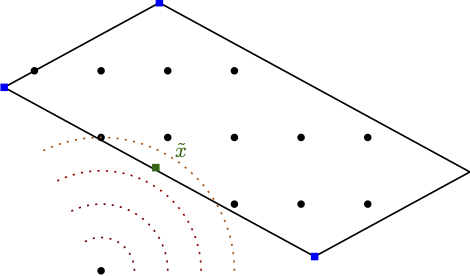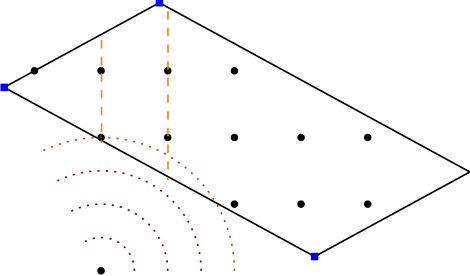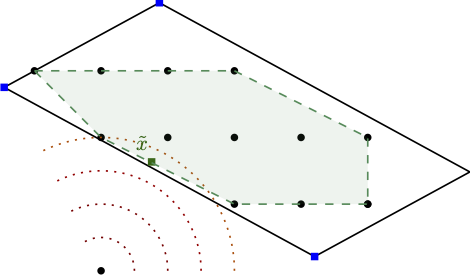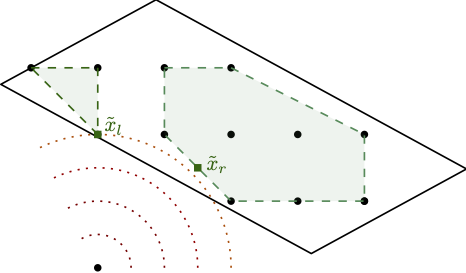Figure 1. Solving stronger subproblems, directly optimizing over the mixed-integer hull can be very powerful. (left) baseline and fractional optimal solution, (middle-left) branching on fractional variable leads only to minor improvement, (middle-right) direct optimization over the mixed-integer hull and fractional solution over the mixed-integer hull, (right) branching once results in optimal solution.

As such, our approach is a Mixed-Integer Conditional Gradient Algorithm that combines a specific Frank-Wolfe algorithm, the Blended Pairwise Conditional Gradient (BPCG) approach of [TTP] (see also the earlier BPCG post), with a branch-and-bound scheme, and solves MIPs as LMOs. Our approach combines several very powerful improvements from conditional gradients with state-of-the-art MIP techniques to obtain a fast algorithm.

### Tricks to make it work

• Leveraging MIP improvements. As our LMOs in the FW subsolver are standards MIPs we can exploit the whole toolbox of MIP solver improvements. In particular, we can use solution pools to collect and reuse previously identified feasible solution. Note, that as our feasible region is never modified (in contrast to, e.g., outer approximation relying on epigraph formulations), all discovered primal solution are globally feasible. In future releases we will also support more advanced reoptimization features of modern MIP solvers, such as carrying over certain presolve information, propagation, and cutting planes.
• Incomplete resolution of nodes. We do not have to solve nodes to near-optimality but rather we can use an adaptive gap strategy to only partially resolve subproblems, significantly reducing the number of iterations in the FW subsolver.
• Warmstarting. We can warmstart the FW subsolver from the run before branching, further cutting down required iterations. For this we can efficiently (as in: for free) write the parent solution as a convex combination of two distinct solutions valid for the left and the right branch, respectively.
• Lazification and blending. We generalize both the lazification [BPZ] and blending approach [BPTW] to apply to the whole tree allowing to aggressively reuse previously found primal feasible solutions. In particular, no primal feasible solution has to be computed or discovered twice.
• Hybrid branching strategy. Finally, we developed a hybrid branching strategy that can further reduce the number of required nodes; however this is highly dependend on the instance.

Combining and exploiting all these things, this results in having only to solve something like 5-8 LMOs calls per node on average and the longer the run and the deeper the tree, the smaller this number is. Asymptotically the average number of LMO calls is approaching something close to 1 as no MIP-feasible solution is computed twice as mentioned above. For a more complex instance, the overall impact of these tricks is shown in Figure 2 below in terms of the average number of LMO calls per node.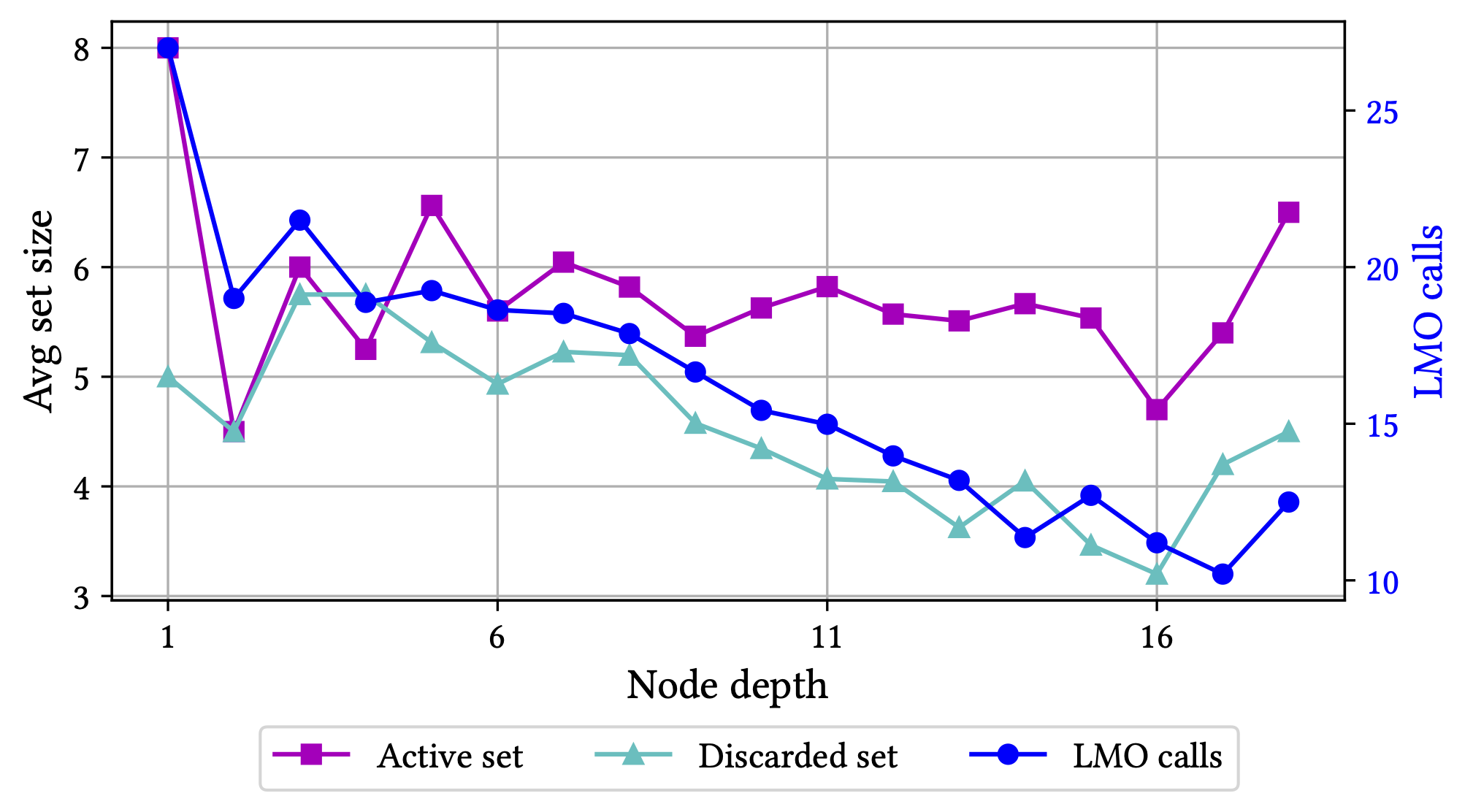Figure 2. Minimizing a quadratic over a cardinality constrained variant of the Birkhoff polytope. Key statistics as a function of the node depth. Both the size of the active (vertex) set and the discarded (vertex) set remain small throughout the run of the algorithm and the average number of LMO calls required to solve a subproblem drops significantly as a function of depth as previously discovered solutions cut out unnecessary LMO calls.

For more details see the preprint [HTBP].

### Implementation of Boscia.jl in a nutshell

• Released under MIT license. Do whatever you want with it and consider contributing to the code base.
• Uses our earlier FrankWolfe.jl Julia package as well the Bonobo.jl branch-and-bound Julia package.
• Uses the Blended Pairwise Conditional Gradient (BPCG) algorithm [TTP] together with (a modified variant of) the adaptive step-size strategy of [PNAJ] from the FrankWolfe.jl package.
• Supports a wide variety of MIP solvers through the MathOptInterface (MOI). Currently, we use SCIP.jl with SCIP 8 in our examples.
• via MOI reads .mps and .lp files out of the box allowing to easily replace linear objectives by convex objectives.
• Interface is identical to that of FrankWolfe.jl: specify the objective and its gradients and provide an LMO for the feasible region.

### Contribute

Most certainly there will be still bugs, calibration issues, and numerical issues in the solver. Any feedback, bug reports, issues, PRs are highly welcome on the package’s github repository.

### A simple example

using Boscia
using FrankWolfe
using Random
using SCIP
using LinearAlgebra
import MathOptInterface
const MOI = MathOptInterface

n = 6

const diffw = 0.5 * ones(n)

##############################
# defining the LMO and using
# SCIP as solver for the LMO
##############################

o = SCIP.Optimizer()

MOI.set(o, MOI.Silent(), true)

x = MOI.add_variables(o, n)

for xi in x
end

lmo = FrankWolfe.MathOptLMO(o) # MOI-based LMO

##############################
# defining objective and
##############################

function f(x)
return sum(0.5*(x.-diffw).^2)
end

@. storage = x-diffw
end

##############################
# calling the solver
##############################

x, _, result = Boscia.solve(f, grad!, lmo, verbose = true)


The output - which is quite wide - then roughly looks like this:

Boscia Algorithm.

Parameter settings.
Tree traversal strategy: Move best bound
Branching strategy: Most infeasible
Absolute dual gap tolerance: 1.000000e-06
Relative dual gap tolerance: 1.000000e-02
Frank-Wolfe subproblem tolerance: 1.000000e-05
Total number of varibales: 6
Number of integer variables: 0
Number of binary variables: 6

---------------------------------------------------------------------------------------------------------------------------------------------------------------------------------------------------
Iteration       Open          Bound      Incumbent      Gap (abs)      Gap (rel)       Time (s)      Nodes/sec        FW (ms)       LMO (ms)  LMO (calls c)   FW (Its)   #ActiveSet  Discarded
---------------------------------------------------------------------------------------------------------------------------------------------------------------------------------------------------
*          1          2  -1.202020e-06   7.500000e-01   7.500012e-01            Inf   3.870000e-01   7.751938e+00            237              2              9         13            1          0
100         27   6.249998e-01   7.500000e-01   1.250002e-01   2.000004e-01   5.590000e-01   2.271914e+02              0              0            641          0            1          0
127          0   7.500000e-01   7.500000e-01   0.000000e+00   0.000000e+00   5.770000e-01   2.201040e+02              0              0            695          0            1          0
---------------------------------------------------------------------------------------------------------------------------------------------------------------------------------------------------

Postprocessing

Blended Pairwise Conditional Gradient Algorithm.
MEMORY_MODE: FrankWolfe.InplaceEmphasis() STEPSIZE: Adaptive EPSILON: 1.0e-7 MAXITERATION: 10000 TYPE: Float64
GRADIENTTYPE: Nothing LAZY: true lazy_tolerance: 2.0
[ Info: In memory_mode memory iterates are written back into x0!

----------------------------------------------------------------------------------------------------------------
Type     Iteration         Primal           Dual       Dual Gap           Time         It/sec     #ActiveSet
----------------------------------------------------------------------------------------------------------------
Last             0   7.500000e-01   7.500000e-01   0.000000e+00   1.086583e-03   0.000000e+00              1
----------------------------------------------------------------------------------------------------------------
PP             0   7.500000e-01   7.500000e-01   0.000000e+00   1.927792e-03   0.000000e+00              1
----------------------------------------------------------------------------------------------------------------

Solution Statistics.
Solution Status: Optimal (tree empty)
Primal Objective: 0.75
Dual Bound: 0.75
Dual Gap (relative): 0.0

Search Statistics.
Total number of nodes processed: 127
Total number of lmo calls: 699
Total time (s): 0.58
LMO calls / sec: 1205.1724137931035
Nodes / sec: 218.96551724137933
LMO calls / node: 5.503937007874016


[TTP] Tsuji, K., Tanaka, K. I., & Pokutta, S. (2021). Sparser kernel herding with pairwise conditional gradients without swap steps. to appear in Proceedings of ICML. arXiv preprint arXiv:2110.12650. pdf

[PNAJ] Pedregosa, F., Negiar, G., Askari, A., & Jaggi, M. (2020, June). Linearly convergent Frank-Wolfe with backtracking line-search. In International Conference on Artificial Intelligence and Statistics (pp. 1-10). PMLR. pdf

[BDRT] Buchheim, C., De Santis, M., Rinaldi, F., & Trieu, L. (2018). A Frank–Wolfe based branch-and-bound algorithm for mean-risk optimization. Journal of Global Optimization, 70(3), 625-644. pdf

[HTBP] Hendrych, D., Troppens, H., Besançon, M., & Pokutta, S. (2022). Convex integer optimization with Frank-Wolfe methods. arXiv preprint arXiv:2208.11010. pdf

[BPTW] Braun, G., Pokutta, S., Tu, D., & Wright, S. (2019, May). Blended conditonal gradients. In International Conference on Machine Learning (pp. 735-743). PMLR. pdf

[BPZ] Braun, G., Pokutta, S., & Zink, D. (2017, July). Lazifying conditional gradient algorithms. In International conference on machine learning (pp. 566-575). PMLR. pdf

]]>
Acceleration of Frank-Wolfe algorithms with open loop step-sizes2022-07-05T01:00:00+02:002022-07-05T01:00:00+02:00http://www.pokutta.com/blog/research/2022/07/05/open_loop_fw-abstractTL;DR: This is an informal discussion of our recent paper Acceleration of Frank-Wolfe algorithms with open loop step-sizes by Elias Wirth, Thomas Kerdreux, and Sebastian Pokutta. In the paper, we study accelerated convergence rates for the Frank-Wolfe algorithm (FW) with open loop step-size rules, characterize settings for which FW with open loop step-size rules is non-asymptotically faster than FW with line search or short-step, and provide a partial answer to an open question in kernel herding.

Written by Elias Wirth.

### Introduction and motivation

Frank-Wolfe algorithms (FW) [F], see Algorithm 1, are popular first-order methods to solve convex constrained optimization problems of the form

$\min_{x\in \mathcal{C}} f(x),$

where $\mathcal{C}\subseteq\mathbb{R}^d$ is a compact convex set and $f\colon \mathcal{C} \to \mathbb{R}$ is a convex and smooth function. FW and its variants rely on a linear minimization oracle instead of potentially expensive projection-like oracles. Many works have identified accelerated convergence rates under various structural assumptions on the optimization problem and for specific FW variants when using line search or short-step, requiring feedback from the objective function, see, e.g., [GH13, GH15, GM, J, LJ] for an incomplete list of references.

Algorithm 1. Frank-Wolfe algorith (FW) [F]

Input: Starting point $x_0\in\mathcal{C}$, step-size rule $\eta_t \in [0, 1]$.
$\text{ }$ 1: $\text{ }$ for $t=0$ to $T$ do
$\text{ }$ 2: $\quad$ $p_t\in \mathrm{argmin}_{p\in \mathcal{C}} \langle \nabla f(x_t), p - x_t\rangle$
$\text{ }$ 3: $\quad$ $x_{t+1} \gets (1-\eta_t) x_t + \eta_t p_t$
$\text{ }$ 4: $\text{ }$ end for

However, little is known about accelerated convergence regimes utilizing open loop step-size rules, a.k.a. FW with pre-determined step-sizes, which are algorithmically extremely simple and stable. In our paper, most of the open loop step-size rules are of the form

$\eta_t = \frac{4}{t+4}.$

One of the main motivations for studying FW with open loop step-size rules is an unexplained phenomenon in kernel herding: In the right plot of Figure 3 of [BLO], the authors observe that FW with open loop step-size rules converges at the optimal rate [BLO] of $\mathcal{O}(1/t^2)$, whereas FW with line search or short-step converges at a rate of $\Omega(1/t)$. For this setting, FW with open loop step-size rules converges at the optimal rate, whereas FW with line search or short-step converge at a suboptimal rate. Despite substantial research interest in the connection between FW and kernel herding, so far, this behaviour remained unexplained.

However, kernel herding is not the only problem setting for which FW with open loop step-size rules can converge faster than FW with line search or short-step. In [B], the author proves that when the feasible region is a polytope, the objective function is strongly convex, the optimum lies in the interior of an at least one-dimensional face of the feasible region $\mathcal{C}$, and some other mild assumptions are satisfied, FW with open loop step-size rules asymptotically converges at a rate of $\mathcal{O}(1/t^2)$. Combined with the convergence rate lower bound of $\Omega(1/t^{1+\epsilon})$ for any $\epsilon > 0$ for FW with line search or short-step [W], this characterizes a setting for which FW with open loop step-size rules converges asymptotically faster than FW with line search or short-step.

### Contributions

The main goal of the paper is to address the current gaps in our understanding of FW with open loop step-size rules.

1. Accelerated rates depending on the location of the unconstrained optimum. For FW with open loop step-size rules, the primal gap does not decay monotonously, unlike for FW with line search or short-step. We thus derive a different proof template that captures several of our acceleration results: For FW with open loop step-size rules, we derive convergence rates of up to $\mathcal{O}(1/t^2)$

1. when the feasible region is uniformly convex and the optimum lies in the interior of the feasible region,
2. when the objective satisfies a Hölderian error bound (think, relaxation of strong convexity) and the optimum lies in the exterior of the feasible region,
3. and when the feasible region is uniformly convex and the objective satisfies a Hölderian error bound.

2. FW with open loop step-size rules can be faster than with line search or short-step. We derive a non-asymptotic version of the accelerated convergence result in [B]. More specifically, we show that when the feasible region is a polytope, the optimum lies in the interior of an at least one-dimensional face of $\mathcal{C}$, the objective is stronly convex, and some additional mild assumptions are satisfied, FW with open loop step-size rules converges at a non-asymptotic rate of $O(1/t^2)$. Combined with the convergence rate lower bound for FW with line search or short-step [W], we thus characterize problem instances for which FW with open loop step-size rules converges non-asymptotically faster than FW with line search or short-step.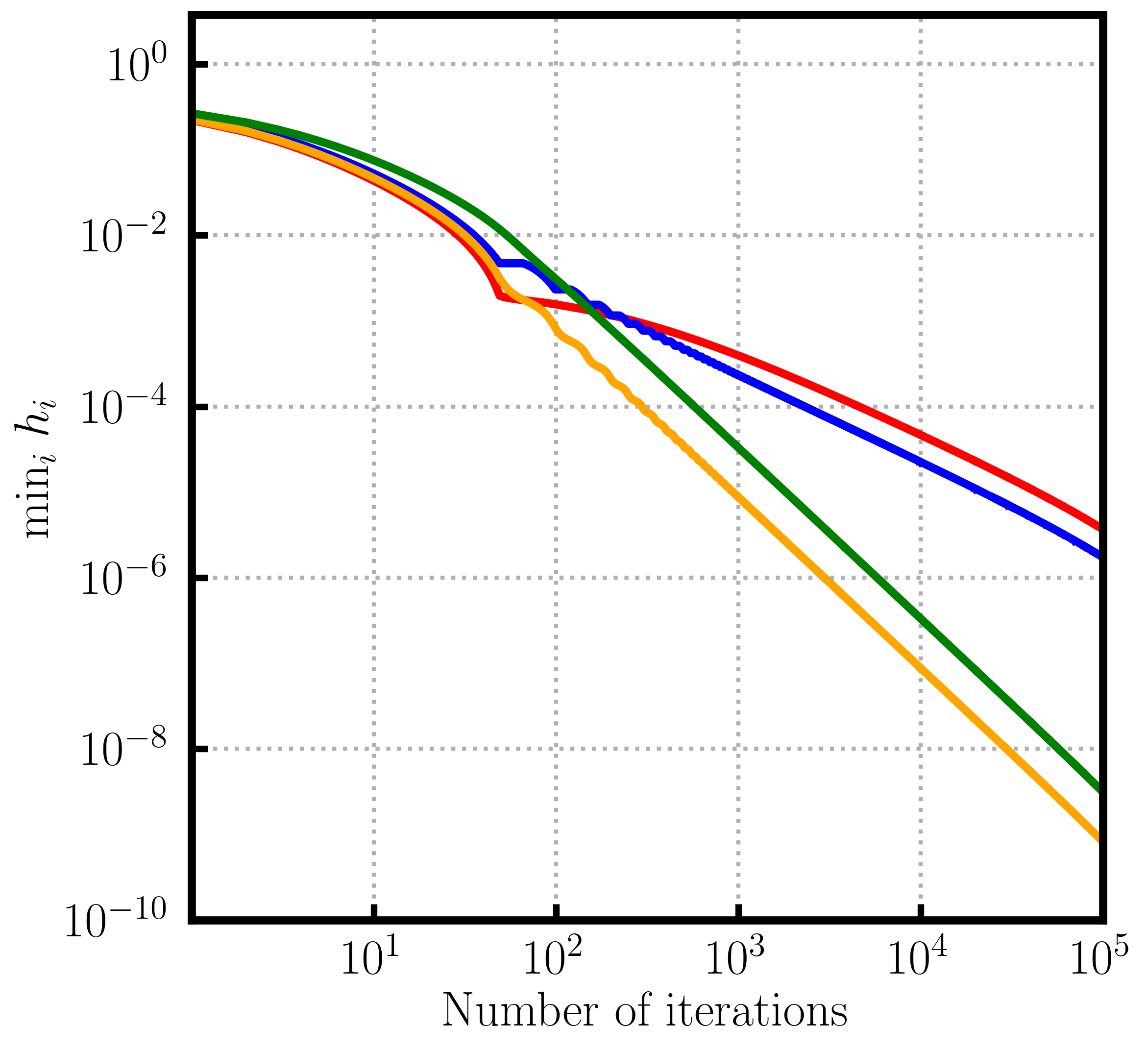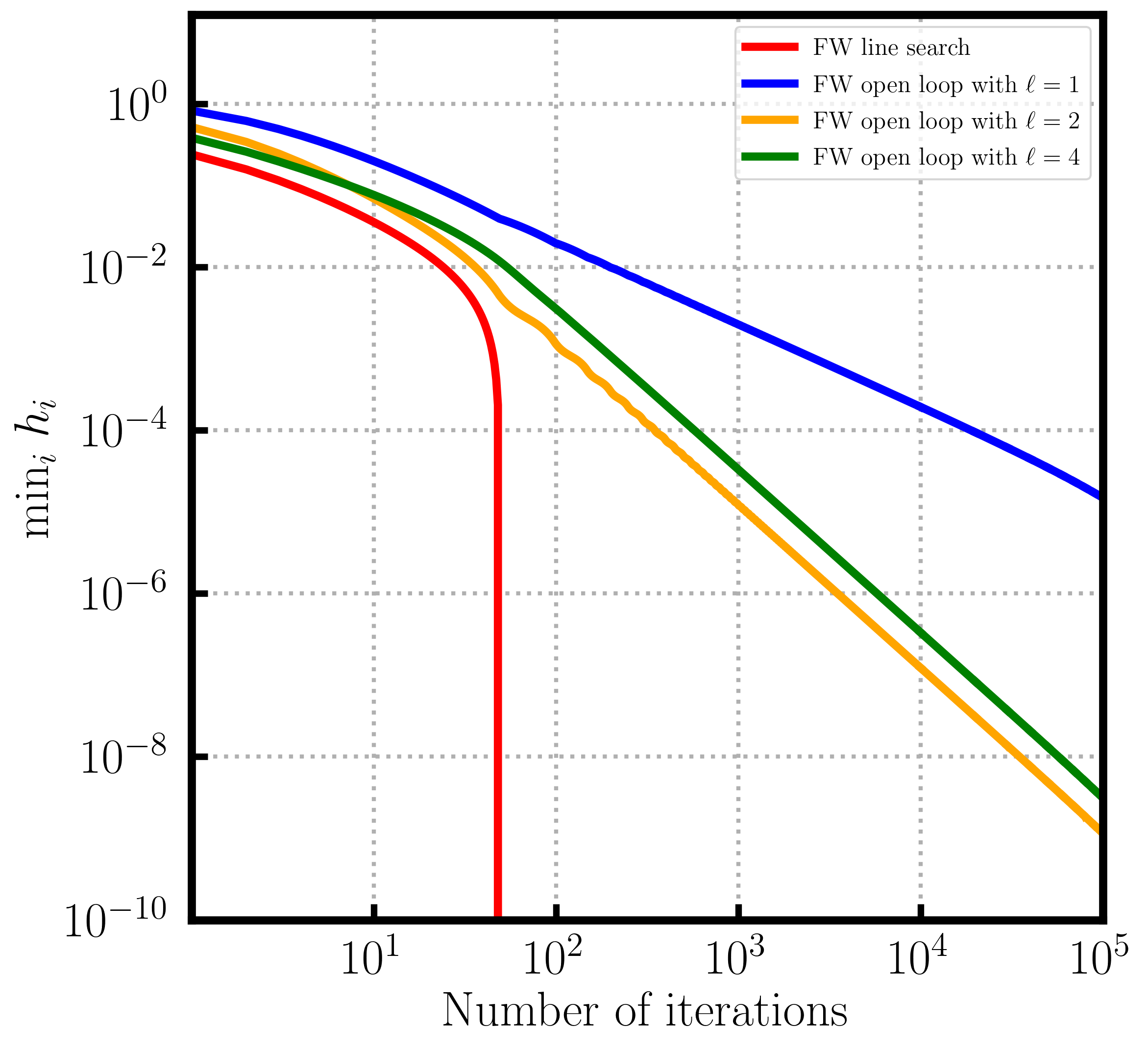Figure 1. Convergence over probability simplex. Depending on the position of the contrained optimum line search (left) can be slower than open loop or (right) faster than open loop.

3. Algorithmic variants. When the feasible region is a polytope, we also study FW variants that were traditionally used to overcome the convergence rate lower bound [W] for FW with line search or short-step. Specifically, we present open loop versions of the Away-Step Frank-Wolfe algorithm (AFW) [LJ] and the Decomposition-Invariant Frank-Wolfe algorithm (DIFW) [GH13]. For both algorithms, we derive convergence rates of order $O(1/t^2)$.

4. Addressing an unexplained phenomenon in kernel herding. We answer the open problem from [BLO], that is, we explain why FW with open loop step-size rules converges at a rate of $\mathcal{O}(1/t^2)$ in the infinite-dimensional kernel herding setting of the right plot of Figure 3 in [BLO].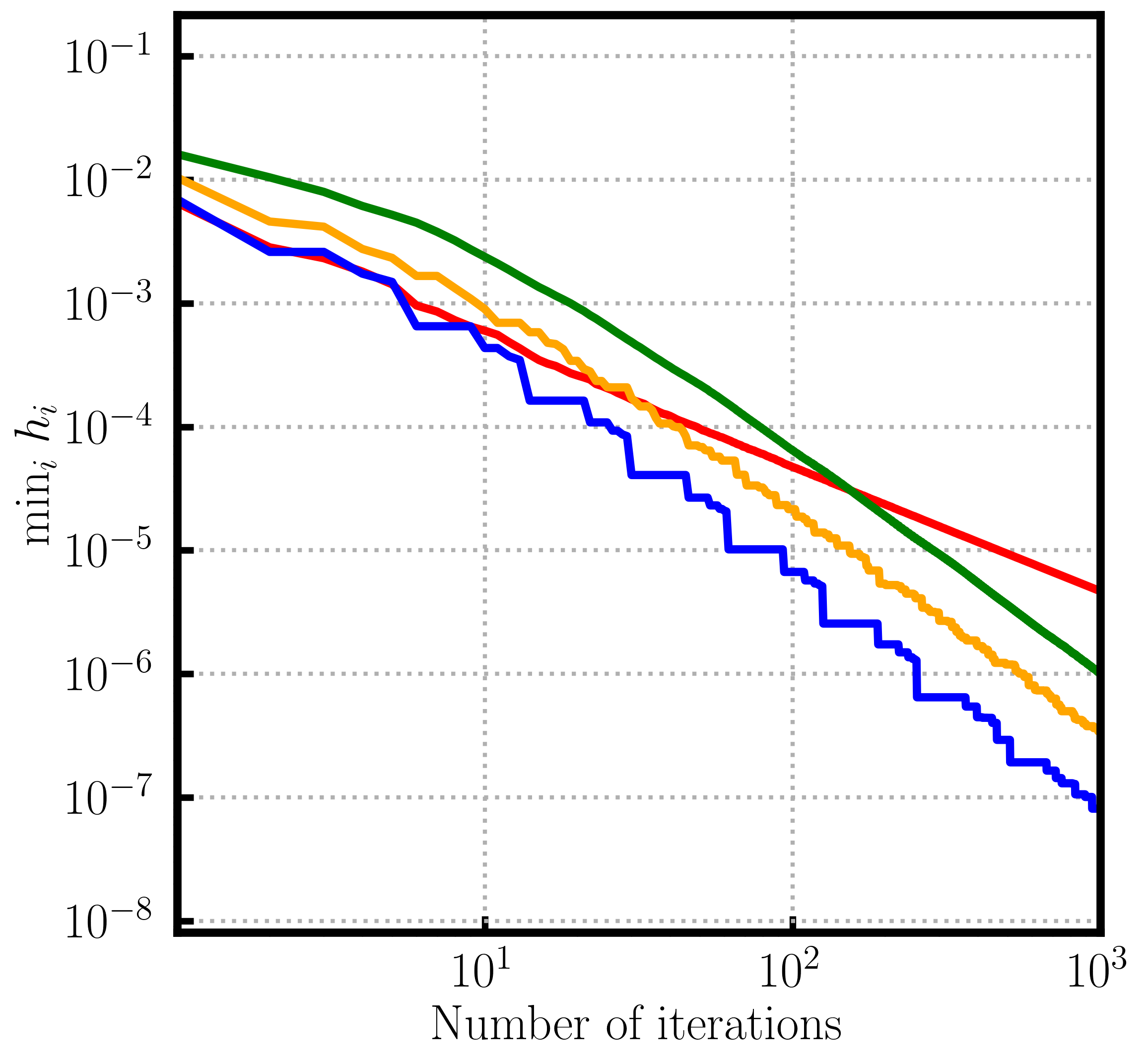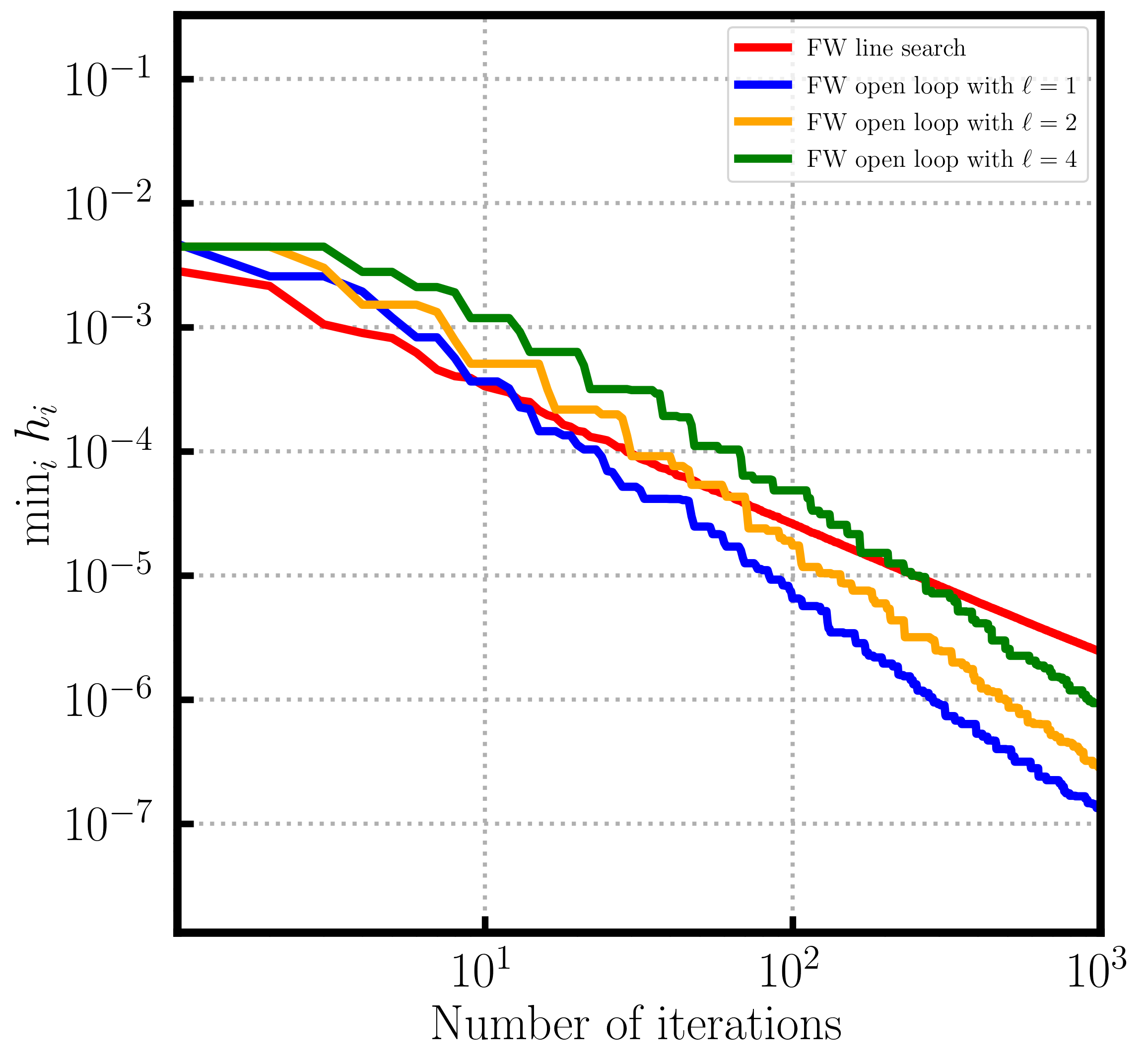Figure 2. Kernel Herding. Open loop step sizes can outperform line search (and short step) (left) uniform (right) non-uniform case.

5. Improved convergence rate after finite burn-in. For many of our results, so as to not contradict the convergence rate lower bound of [J], the derived accelerated convergence rates only hold after an initial number of iterations, that is, the accelerated rates require a burn-in phase. This phenomenon is also referred to as accelerated local convergence [CDLP, DCP]. We study this behvaviour both in theory and with numerical experiments for FW with open loop step-size rules.

### References

[B] Bach, F. (2021). On the effectiveness of richardson extrapolation in data science. SIAM Journal on Mathematics of Data Science, 3(4):1251–1277.

[BLO] Bach, F., Lacoste-Julien, S., and Obozinski, G. (2012). On the equivalence between herding and conditional gradient algorithms. In ICML 2012 International Conference on Machine Learning.

[CDLP] Carderera, A., Diakonikolas, J., Lin, C. Y., and Pokutta, S. (2021a). Parameter-free locally accelerated conditional gradients. arXiv preprint arXiv:2102.06806.

[DCP] Diakonikolas, J., Carderera, A., and Pokutta, S. (2020). Locally accelerated conditional gradients. In International Conference on Artificial Intelligence and Statistics, pages 1737–1747. PMLR.

[F] Frank, M., Wolfe, P., et al. (1956). An algorithm for quadratic programming. Naval research logistics quarterly, 3(1-2):95–110

[GH13] Garber, D. and Hazan, E. (2013). A linearly convergent conditional gradient algorithm with applications to online and stochastic optimization. arXiv preprint arXiv:1301.4666.

[GH15] Garber, D. and Hazan, E. (2015). Faster rates for the frank-wolfe method over strongly-convex sets. In International Conference on Machine Learning, pages 541–549. PMLR.

[GM] Guélat, J. and Marcotte, P. (1986). Some comments on wolfe’s ‘away step’. Mathematical Programming, 35(1):110–119.

[J] Jaggi, M. (2013). Revisiting frank-wolfe: Projection-free sparse convex optimization. In International Conference on Machine Learning, pages 427–435. PMLR.

[LJ] Lacoste-Julien, S. and Jaggi, M. (2015). On the global linear convergence of frank-wolfe optimization variants. Advances in Neural Information Processing Systems, 28:496–504.

[W] Wolfe, P. (1970). Convergence theory in nonlinear programming. Integer and nonlinear programming, pages 1–36.

]]>
Elias Wirth
Pairwise Conditional Gradients without Swap Steps2022-05-21T07:00:00+02:002022-05-21T07:00:00+02:00http://www.pokutta.com/blog/research/2022/05/21/bpcg-abstractTL;DR: This is an informal summary of our recent article Sparser Kernel Herding with Pairwise Conditional Gradients without Swap Steps by Kazuma Tsuji, Ken’ichiro Tanaka, and Sebastian Pokutta, which was accepted at ICML 2022. In this article we present a modification of the pairwise conditional gradient algorithm that removes the dreaded swap steps. The resulting algorithm can even be applied to infinite dimensional feasible regions and promotes high levels of sparsity, which is useful in applications such as e.g., kernel herding.

Written by Sebastian Pokutta.

## What is the paper about and why you might care

One of the most appealing conditional gradient algorithm is the Pairwise Conditional Gradient (PCG) algorithm introduced in [LJ] as a modification of the Away-step Frank-Wolfe (AFW) algorithm. The Pairwise Conditional Gradient algorithm essentially performs a normal Frank-Wolfe step and an away-step simultaneously. This gives much higher convergence steps in practice, however in the analysis now, so-called swap steps appear, where weight is shifted from an away-vertex to a new Frank-Wolfe vertex. For these steps, we cannot bound the primal progress and additionally, there are potentially lots of these steps, so that the theoretical convegence bounds are much worse than what we observe in practice. In fact its guarantees are worse than the guarantees for the Away-step Frank-Wolfe algorithm, that is almost always outperformed by the Pairwise Conditional Gradient algorithm in practice. Various modifications of PCG had been suggested, e.g., in [RZ] and [MGP] to deal with this issue, however often requiring subprocedures whose costs cannot be easily bounded.

It should also be mentioned that the PCG algorithm is particularly nice in the case of polytopes and almost becomes a combinatorial algorithm as the resulting directions are always formed by direction arising from the line-segment between two vertices and thus there are only finitely many such directions.

## Our results

By borrowing machinery from [BPTW], we show that with a minor modification of the PCG algorithm we can avoid drop steps altogether. In a nutshell, the idea is to limit the pairwise directions to those formed by FW vertices and away-vertices from the current active set and only if those steps are not good enough we perform a normal FW step. This way swap steps cannot appear anymore however we require a key technical lemma that shows that the reduced pairwise steps are still good enough. We call this algorithm the Blended Pairwise Conditional Gradient (BPCG) algorithm; see below.

The resulting algorithm has a theoretical convergence rate that is basically the same as the one for the AFW algorithm (up to small constant factors). In fact, it inherits all convergence proofs that hold for the AFW algorithm. Moreover, it exhibits the same convergence speed as (even often faster than) the original PCG algorithm in practice. The algorithm also works in the infinite dimensional setting, which is not true for the original PCG algorithm due to the dimension dependence arising from the number of swap steps in the convergence rate. The iterates produced by BPCG are very sparse, where sparsity is measured in the number of elements in the convex combinations of the iterates making it very suitable, e.g., for kernel herding.

Blended Pairwise Conditional Gradient Algorithm (BPCG); slightly simplified
Input: Smooth convex function $f$ with first-order oracle access, feasible region (polytope) $P$ with linear optimization oracle access, initial vertex $x_0 \in P$.
Output: Sequence of points $x_0, \dots, x_{T}$
$$S_0 = \{x_0\}$$
For $t = 0, \dots, T-1$ do:
$\quad a_t \leftarrow \arg\max_{v \in S_t} \langle \nabla f(x_{t}), v \rangle$ $\qquad$ {Away-vertex over $S_t$}
$\quad s_t \leftarrow \arg\min_{x \in S_t} \langle \nabla f(x_{t}), v \rangle$ $\qquad$ {Local FW-vertex over $S_t$}
$\quad w_t \leftarrow \arg\min_{x \in P} \langle \nabla f(x_{t}), v \rangle$ $\qquad$ {(Global) FW-vertex over $P$}
$\quad$ If $$\langle \nabla f(x_{t}), a_t - s_t \rangle \geq \langle \nabla f(x_{t}), x_t - w_t \rangle$$: $\qquad$ {Local gap as large as global gap}
$\quad\quad$ $d_t = a_t - s_t$ $\qquad$ {Pick (local) pairwise direction}
$\quad\quad$ $x_{t+1} \leftarrow x_t - \gamma_t d_t$ via line search s.t. residual weight of $a_t$ nonnegative
$\quad\quad$ If $a_t$ removed then {Drop step} $$S_{t+1} \leftarrow S_t \setminus \{a_t\}$$ else {Descent step} $$S_{t+1} \leftarrow S_t$$
$\quad$ Else $\qquad$ {Normal FW Step}
$\quad\quad$ $d_t = x_t - w_t$ $\qquad$ {Pick FW direction}
$\quad\quad$ $x_{t+1} \leftarrow x_t - \gamma_t d_t$ via line search with $\gamma_t \in [0,1]$
$\quad\quad$ Update $$S_{t+1} \leftarrow S_t \cup \{w_t\}$$

The key to the convergence proofs is the following lemma that show that these local pairwise steps combined with global FW steps are good enough to ensure sufficient progress per iteration

Key Lemma. In each iteration $t$ it holds: $2 \langle \nabla f(x_{t}), d_t \rangle \geq \langle \nabla f(x_{t}), a_t - w_t \rangle.$

For those in the know this is all you need to prove convergence: the term on the right-hand side is the strong Wolfe gap and as such progress from smoothness with $d_t$ can be lower bounded by the progress from smoothness with the strong Wolfe gap. See e.g., Cheat Sheet: Smooth Convex Optimization and Cheat Sheet: Linear convergence for Conditional Gradients (towards the end when analyzing AFW) to understand how one continues from here. Also similarly, with this inequality we can apply e.g., the reasoning in Cheat Sheet: Hölder Error Bounds (HEB) for Conditional Gradients to extend the results, to e.g., sharp functions etc.

### Computations

We also performed several computational experiments to evaluate the performance of BPCG and in fact the algorithm has been also implemented in the FrankWolfe.jl Julia Package (See this post or [BCP]) and is now the recommended default active-set based conditional gradient algorithm. With a simple trick by adding a factor on the left-hand side in front of the gap test in the algorithm one can further improve sparsity; see the original paper for more details.

Below in Figure 1, we provide a simple convergence test for the approximate Carathéodory problem. We can see that in iterations BPCG is basically identical to PCG (as expected) however in time it is faster as the local updates are often cheaper.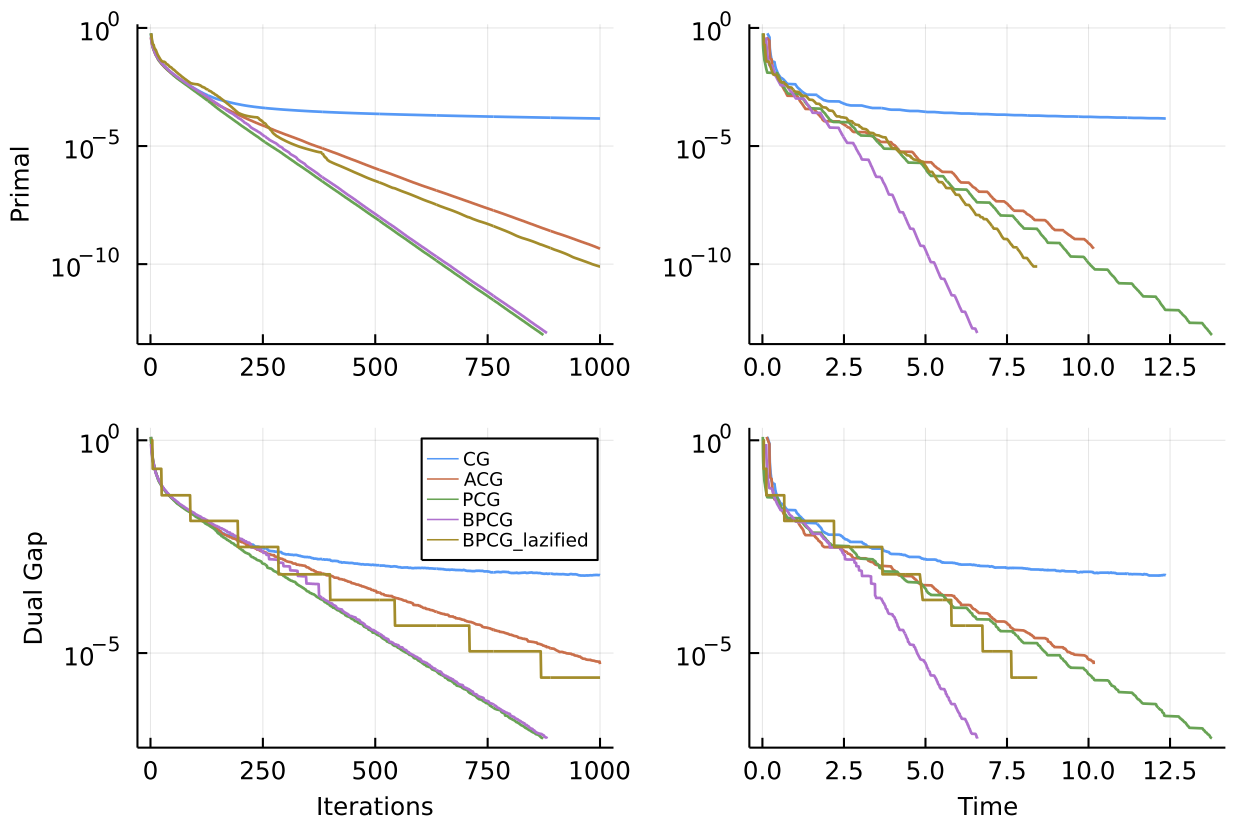Figure 1. Convergence on approximate Carathéodory instance over polytope of dimension $n=200$.

#### Sparsity

While the convergence plot above is quite typical and in terms of speed per iteration or wallclock time BPCG is usually at least as good as PCG (and sometimes faster), the real advantage is often in terms of sparsity as the preference for local steps promotes sparsity. This can be seen in the two plots below.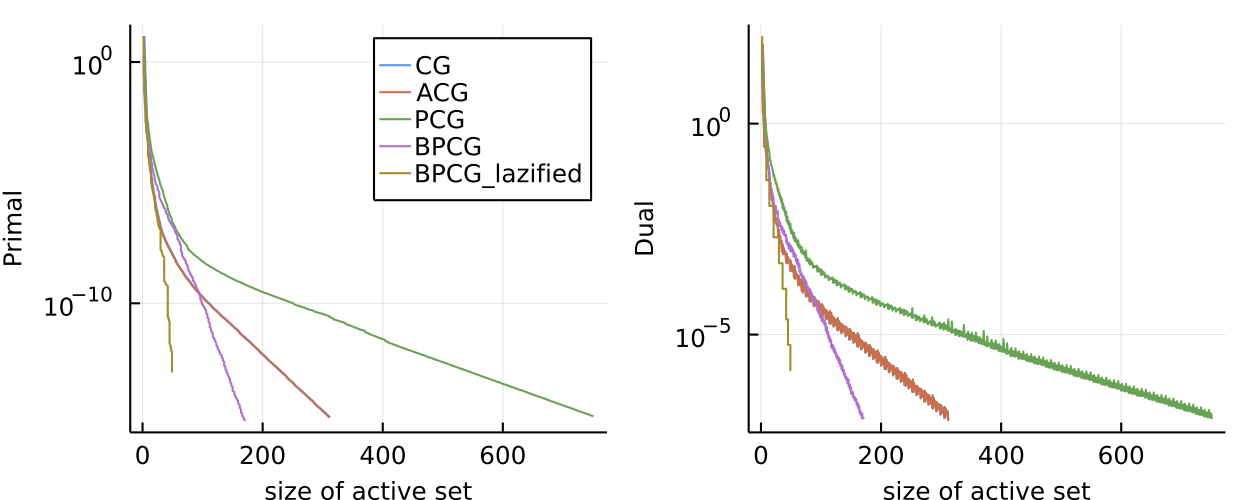Figure 2. Sparse regression problem over $l_5$-norm ball. Here we plot primal value and dual gap vs. size of the active set. BPCG consistently delivers smaller primal and dual values for the same number of atoms in the active set.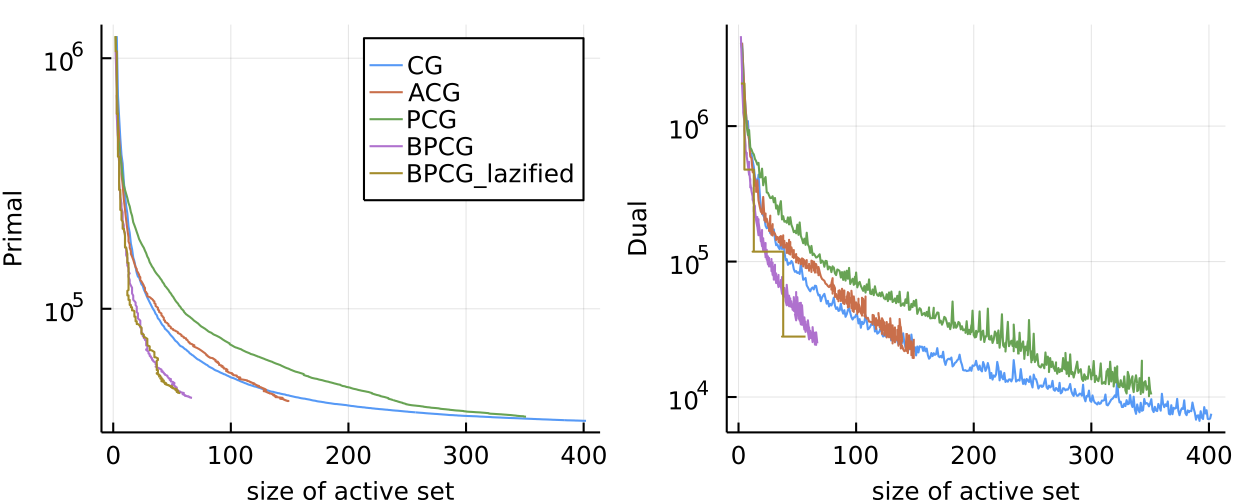Figure 3. Movielens matrix completion problem. Same logic as above with similar results.

#### Kernel Herding

Finally we also considered various kernel herding problems. Here are two examples; the graphs are a little packed.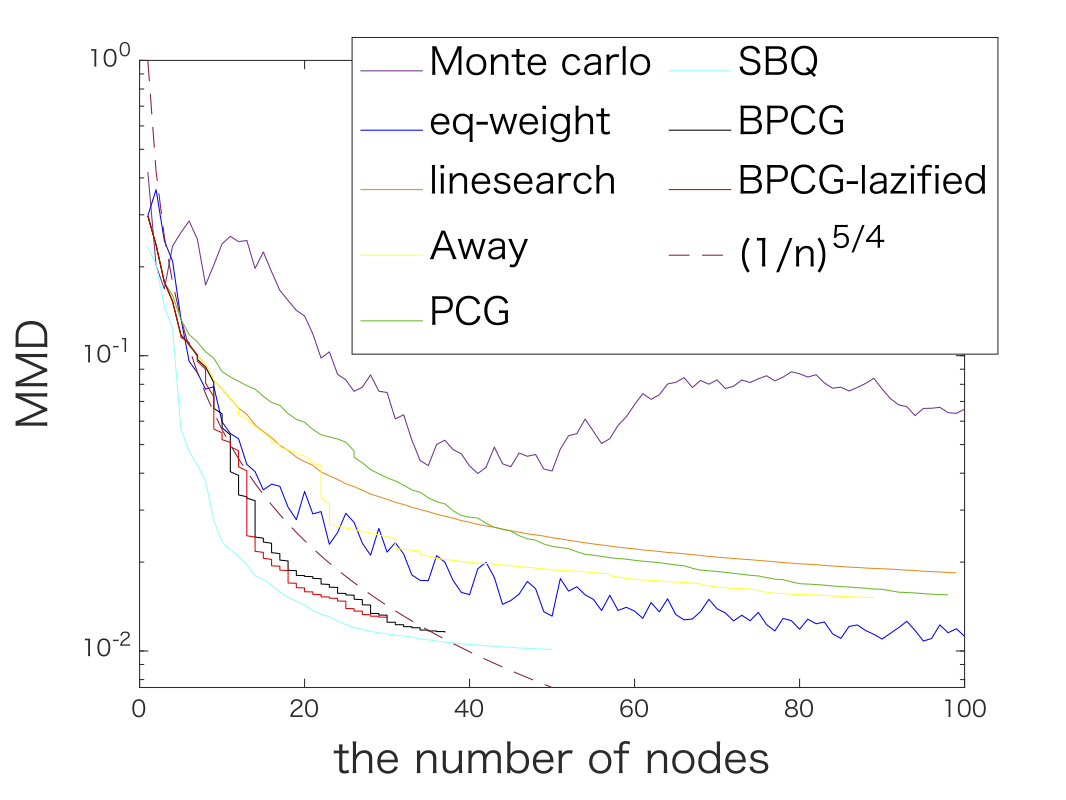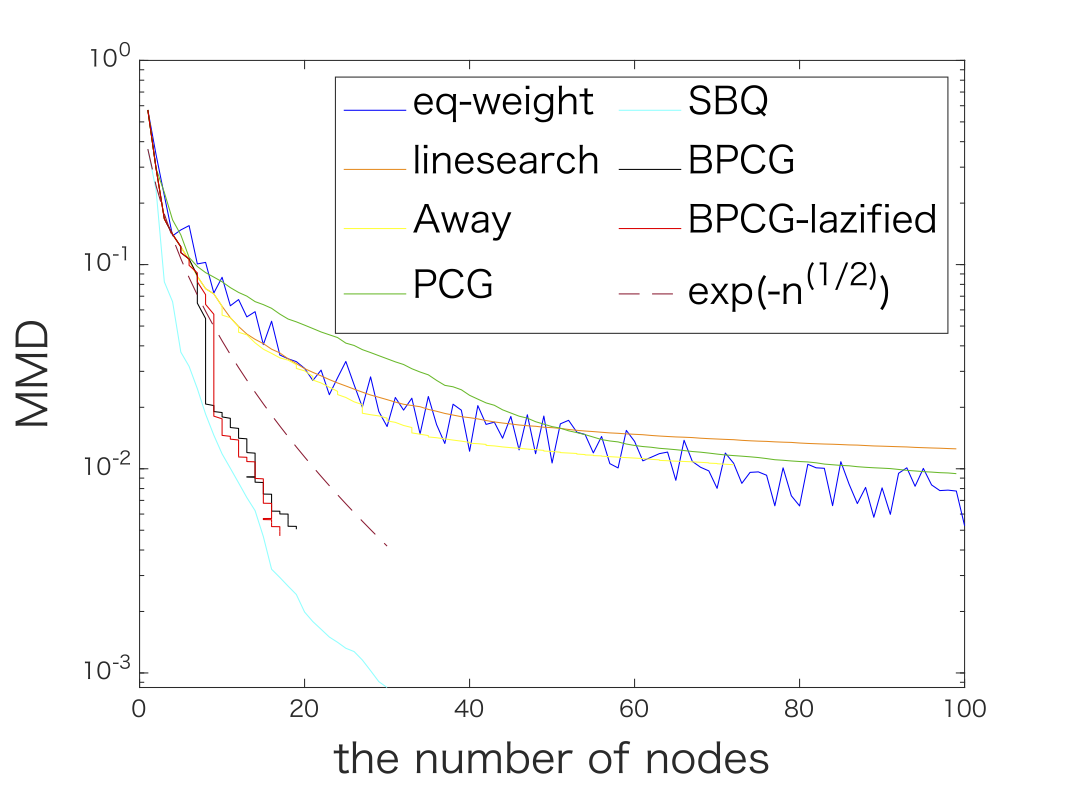Figure 4. Kernel herding for Matérn kernel (left) and Gaussian kernel (right). In both cases BPCG delivers results on par with the Sequential Bayesian Quadrature method (SBQ) however at the fraction of the cost.

### References

[LJ] Lacoste-Julien, S., & Jaggi, M. (2015). On the global linear convergence of Frank-Wolfe optimization variants. In Advances in Neural Information Processing Systems (pp. 496-504). pdf

[RZ] Rinaldi, F., & Zeffiro, D. (2020). A unifying framework for the analysis of projection-free first-order methods under a sufficient slope condition. arXiv preprint arXiv:2008.09781. pdf

[MGP] Mortagy, H., Gupta, S., & Pokutta, S. (2020). Walking in the shadow: A new perspective on descent directions for constrained minimization. Advances in Neural Information Processing Systems, 33, 12873-12883. pdf

[BPTW] Braun, G., Pokutta, S., Tu, D., & Wright, S. (2019, May). Blended conditonal gradients. In International Conference on Machine Learning (pp. 735-743). PMLR. pdf

[BCP] Besançon, M., Carderera, A., & Pokutta, S. (2022). FrankWolfe. jl: A High-Performance and Flexible Toolbox for Frank–Wolfe Algorithms and Conditional Gradients. INFORMS Journal on Computing. pdf

]]>
Sebastian Pokutta
Quantum Computing for the Uninitiated: The Basics2022-05-07T06:00:00+02:002022-05-07T06:00:00+02:00http://www.pokutta.com/blog/research/2022/05/07/cheatsheet-quantum-computing-basicsTL;DR: Cheat Sheet for Quantum Computing. Target audience is non-physicists and no physics background required. This is the very first post in the series presenting the very basics to get started. Long and technical.

Posts in this series (so far).

My apologies for incomplete references—this should merely serve as an overview.

This will be a series on quantum computing. Our perspective here will be a more mathematical or computer science one. I am not a physicist so I will not be able to provide sophisticated physical interpretations. Nonetheless, I will try to provide physics context here and there to highlight the difficulties when going from the rather abstract mathematical formalism of quantum mechanics and quantum computing to the real (physical) world, which leads to many challenging—sometimes philosophical—problems. Feel free to comment if you have suggestions for improvements.

In this first installment we will really just look at the basics of quantum computing and I end this post with a famous motivating example showing the power of quantum mechanics. Most of what we are going to see today is linear algebra with Dirac notation; consider this a warm-up to get used to the notation as well as a refresh on linear algebra basics in the context of quantum mechanics, which is the basis for quantum computing. For a more extensive introduction, check out [dW19], [M07], and [P21] which I heavily relied upon and from where some of the examples are taken. I also extensively used wikipedia, which has quite accessible articles on most of the basic stuff that we will see today.

## Dirac notation

We will be working in Hilbert spaces over complex numbers. A very useful notation in quantum mechanics is the Dirac notation (also called: bra-ket notation), which is used to write quantum states, which in turn are nothing else but special vectors in that Hilbert space. Slightly abusing notions, following Dirac’s original intent, basically an element $\phi \in \mathcal H$ on the primal side is a ket, written as $\ket{\phi}$ and corresponds to a column vector and an element $\psi \in \mathcal H$ on the dual side is a bra, written as $\bra{\psi}$, corresponding to a row vector. This notation has many advantages as it ensures that we automatically distinguish between primal and dual and the inner product follows naturally; we will see all this in a second.

### ket and bra

Usually we will have an orthonormal basis (say, $\ket{0}, \dots, \ket{N-1}$ of $N$ vectors) that generates our Hilbert space $\mathcal H = \langle \ket{0}, \dots, \ket{N-1} \rangle$ and each element $\ket{\phi} \in \mathcal H$ (abusing notation here), is given by:

$\ket{\phi} = \sum_{i = 0}^{N-1} \alpha_i \ket{i} \qquad \text{ with } \qquad \alpha_i \in \CC,$

equivalently, due to the standard isomorphism in the finite dimensional case, we can write

$\newcommand\vec{\begin{pmatrix}#1\end{pmatrix}} \ket{\phi} = \vec{\alpha_0 \\ \vdots \\ \alpha_{N-1}},$

and naturally associated with each ket $\ket{\phi}$ is a bra $\bra{\phi}$, which is defined as the conjugate transpose of $\ket{\phi}$:

$\newcommand\vec{\begin{pmatrix}#1\end{pmatrix}} \bra{\phi} = \vec{\alpha_0^\esx, \dots, \alpha_{N-1}^\esx},$

where the $\esx$ denotes the conjugate operation here, mapping a complex number $\alpha = x + iy$ to $\alpha^\esx = x + i (-y) = x - i y$. Note, that the bra-ket notation is simply a different notation for vectors and in particular, it holds:

$\ket{a \phi + b \gamma} = a \ket{\phi} + b \ket{\gamma} \qquad \text{ and } \qquad \bra{a \phi + b \gamma} = a^\esx \bra{\phi} + b^\esx \bra{\gamma}.$

However, the bra notation has the built-in conjugate for its coefficients, which ensures that basically all properties, e.g., of the inner product simply follow from applying the “Euclidean”-style inner product.

### Inner product

With the above we naturally obtain our scalar product as our basis is orthonormal, i.e., $$\braket{i \mid j} = \delta_{ij}$$. To this end, let

$\newcommand\vec{\begin{pmatrix}#1\end{pmatrix}} \ket{\phi} = \vec{\alpha_0 \\ \vdots \\ \alpha_{N-1}} \qquad \text{ and } \qquad \bra{\psi} = \vec{\beta_0^\esx, \dots, \beta_{N-1}^\esx},$

then we have that

$\newcommand\vec{\begin{pmatrix}#1\end{pmatrix}} \braket{\psi \mid \phi} = \vec{\beta_0^\esx, \dots, \beta_{N-1}^\esx} \cdot \vec{\alpha_0 \\ \vdots \\ \alpha_{N-1}} = \sum_{i = 0}^{N-1} \beta_i^\esx \alpha_i,$

where we have exploited the built-in conjugate in the bra.

Properties of $\braket{\psi \mid \phi}$.
a. $\braket{\psi \mid \phi}$ is a Hermitian form, i.e., $\braket{\psi \mid \phi} = \braket{\phi \mid \psi}^\esx$
b. linear in right-hand side: $\braket{\psi \mid a \phi + b \gamma} = a \braket{\psi \mid \phi} + b \braket{\psi \mid \gamma}$
c. anti-linear in left-hand side: $\braket{a \psi + b \delta \mid \phi} = a^\esx \braket{\psi \mid \phi} + b^\esx \braket{\delta \mid \phi}$
d. $\braket{\psi \mid \phi} \in \CC$
e. $\braket{\phi \mid \phi} \in \RR$ and $\braket{\phi \mid \phi} > 0$ iff $\ket{\phi} \neq 0$

We also obtain the squared norm $\norm{\ket{\phi}}^2$

$\norm{\ket{\phi}}^2 = \braket{\phi \mid \phi} =\sum_{i = 0}^{N-1} \alpha_i^* \alpha_i = \sum_{i = 0}^{N-1} \abs{\alpha_i}^2 \in \RR.$

In the following let $A^\dagger$ denote the adjoint of the matrix $A$, which is nothing else but the conjugate transpose of $A$, i.e., $A^\dagger = (A^T)^\esx$. In particular, if $A$ corresponds to multiplication with $z \in \CC$, then $A^\dagger$ corresponds to the multiplication with $z^\esx$. It is useful here to extend the dagger notion also the vectors to render bras and kets dual to each other, i.e., $(\ket{\phi})^\dagger = \bra{\phi}$, which is in line with our definition of the bra as conjugate transpose of the ket. With the general rule that the adjoint of the product is equal to the reverse-order product of the adjoints, most of the below follow naturally; see also [M07] for a broader exposition. For some of those rules, we assume that we will be working with finite dimensional vector spaces.

Useful rules.
a. $\ket{A \phi} = A \ket{\phi}$ and $\bra{A\phi} = \bra{\phi} A^\dagger$.
b. $(A \ket{\phi})^\dagger = (\ket{A \phi})^\dagger = \bra{\phi} A^\dagger$.
c. $A (\alpha \ket{\phi} + \beta \ket{\psi}) = \alpha A \ket{\phi} + \beta A \ket{\psi}$ and $(\alpha \bra{\phi} + \beta \bra{\psi}) A = \alpha \bra{\phi} A + \beta \bra{\psi} A = \alpha \bra{A^\dagger \phi} + \beta \bra{A^\dagger \psi}$.
d. If $U$ is a unitary matrix, i.e., $U^\dagger U = U U^\dagger = I$ then $\braket{\psi \mid \phi} = \braket{U \psi \mid U\phi}$.

## Pure States

With this we can now define what pure (quantum) states are. These are nothing else but linear combinations of elements from our orthonormal basis ${\ket{i}}_{i = 0, \dots, N-1}$.

$\ket{\phi} = \sum_{i = 0}^{N-1} \alpha_i \ket{i} \qquad \text{ with } \qquad \alpha_i \in \CC,$

and additionally we require that

$\norm{\ket{\phi}}^2 = \braket{\phi \mid \phi} =\sum_{i = 0}^{N-1} \alpha_i^* \alpha_i = \sum_{i = 0}^{N-1} \abs{\alpha_i}^2 = 1,$

and hence also $\norm{\ket{\phi}} = 1$.

## Measurements

An important operation that we can apply to a state is a measurement with the aim to extract information from the state.

### Projective Measurements

We first consider so-called projective measurements. To this end, let us briefly recall the definition and properties of an orthogonal projection matrix:

Definition and Properties: Orthogonal projection matrices. A square matrix $P : \mathcal H \rightarrow \mathcal H$ is an orthogonal projection matrix if: $P^2 = P = P^\dagger$ Properties.
a. $\braket{\psi \mid P \phi} = \braket{\psi P \mid \phi}$
b. Eigenvalues of $P$ are $0$ and $1$ only
c. $\norm{\ket{P \phi}}^2 = \braket{P \phi \mid P \phi} = \braket{\phi \mid P^\dagger P \mid \phi} = \braket{\phi \mid P \mid \phi} = \tr(P \ketbra{\phi}{\phi})$. The matrix $\rho = \ketbra{\phi}{\phi}$ here is called density matrix and we will revisit it later.

See also wikipedia for more useful properties. With this we can define the measurement operation:

Definition: Measurement. A measurement with $m$ outcomes is a set of orthogonal projection matrices $P_1, \dots, P_m$ that decompose the identity matrix $I = \sum_{i = 1}^m P_i$.

Note, that the above definition implies that $P_i P_j = 0$ for $i \neq j$: Simply multiply $I = \sum_{i = 1}^m P_i$ with some $P_j$ from the right, then reorder to $0 = P_1P_j + \dots + P_j(P_j - I) + \dots + P_nP_j$. Since the images of two distinct $P_i$ only intersect in $0$ it follows that $P_1P_j = 0$ for all $i \neq j$; full proof left to the interested reader or see e.g., Theorem 2.13 here.

We can now write $\ket{\phi} = I \ket{\phi} = \sum_{i = 1}^m P_i \ket{\phi}$. As $\norm{\ket{\phi}}^2 = 1$ and since the projections are orthogonal, we have that $1 = \sum_{i = 1}^m \norm{P_i \ket{\phi}}^2$, as $P_iP_j = 0$ for $i \neq j$ and $P_i^2 = P_i$, i.e., we obtain a probability distribution. The process of measuring now samples an $i$ according to this probability distribution, i.e., with probability $\norm{\ket{P_i\phi}}^2$ and maps $\ket{\phi} \mapsto \ket{P_i \phi} / \norm{\ket{P_i\phi}}$, which is again a (valid) state. After measuring, the state $\ket{\phi}$ ends up in an eigenstate of the measurement and thus the state changes, except for when $\ket{\phi}$ is already in an eigenstate of the measurement in which case it does not change.

Note that measurements are invariant w.r.t. the global phase, i.e., $\ket{\phi}$ and $e^{ir} \ket{\phi}$ produce the same measurement outcomes and statistics and the obtained states after measurement are also identical up to $e^{ir}$-rotation. In fact the global rotation $e^{ir}$ only affects the phase of the complex coefficients but not their absolute value. This is not to be confused with the relative phase differences in superpositions which are important.

### Measuring in the computational base

Later, we will be mostly concerned with the case where the $P_i$ are given as rank-1 projectors into the actual (computational) basis $\ket{0}, \dots, \ket{N-1}$, i.e., $P_i = \ketbra{i}{i}$. Let $\ket{\phi} = \sum_{i = 0}^{N-1} \alpha_i \ket{i}$. We then have:

$P_j \ket{\phi} = \ketbra{j}{j} \ket{\phi} = \sum_{i = 0}^{N-1} \alpha_i \ketbra{j}{j} \ket{i} = \alpha_j \ket{j},$

we purposefully (only this time) did not clean up bra and ket double separators for the sake of exposition. Thus we obtain that we measure $P_j = \ketbra{j}{j}$ with probability $\norm{\ket{P_j\phi}}^2 = \norm{\alpha_j \ket{j}}^2 = \Abs{\alpha_j}^2$. Alternatively, just for the sake of getting used to the bra-ket notation:

\begin{align*} \norm{\ket{P_j\phi}}^2 & = \norm{\ket{j}\bra{j} \ket{\phi}}^2 = \braket{\phi \mid \ketbra{j}{j} \mid \phi} = \braket{\phi \mid j} \braket{j \mid \phi} \\ & = \braket{j \mid \phi}^\esx \braket{j \mid \phi} = \Abs{\braket{j \mid \phi}}^2 \\ & = \Abs{\sum_{i = 0}^{N-1} \braket{j \mid \alpha_i i}}^2 = \Abs{\sum_{i = 0}^{N-1} \alpha_i \braket{j \mid i}}^2 = \Abs{\alpha_j}^2. \end{align*}

The resulting state after measuring $j$ via $P_j$ is

$\ket{P_j\phi} / \norm{\ket{P_j\phi}} = \frac{\alpha_j}{\Abs{\alpha_j}} \ket{j},$

i.e., when measuring in the computational basis our superposition collapses to a classical state.

The Physics spin: Measurements, collapse of superpositions, and Schrödinger’s cat. While we quite non-chalantly applied our measurements, e.g., by simply multiplying with the projection matrix and renormalization, the physical reality seems to be much more complicated. In fact, up to today it is unclear when exactly the measurement happens that forces the quantum superposition to collapse to a classical state. The famous thought experiment of Schrödinger made this problem very apparent. Simplifying, the box with the cat is built so that the life of a cat in a box is linked one-to-one to a quantum superposition, i.e., it is a mechanism to upscale the effect from the atomic domain to the macroscopic one. Now when does the measurement take place that decides the fate of the cat? When you open the box? What if you can hear the cat being alive in the box? I.e., when exactly does the superposition cease to be a superposition and collapses to a classic state? There are tons of interpretation of quantum mechanics that give different answers to the questions posed by Schrödinger’s cat. The most prevalent one, which also seems to be the most unsatisfying one as it is basically stating the obvious, is the so-called Copenhagen interpretation: “A system stops being a superposition of states and becomes either one or the other when an observation takes place.” Now, what is an “observation”? For further reading check out wikipedia, but beware this easily becomes a rabbit hole.

Note that while we have seen only rank-1 projectors in this section, it is very well possible to also have higher rank projectors. For example consider the state:

$\ket{\phi} = \frac{1}{\sqrt{3}} \ket{1} + \frac{2}{\sqrt{3}} \ket{N},$

and the projectors (assuming $N$ is even)

$P_1 = \sum_{i = 1}^{N/2} \ketbra{i}{i} \qquad \text{and} \qquad P_2 = \sum_{i = N/2 + 1}^{N} \ketbra{i}{i}.$

Clearly, $I = P_1 + P_2$. We measure with the first projector $P_1$ with probability

$\norm{P_1 \ket{\phi}}^2 = \tr(P_1 \ketbra{\phi}{\phi}) = 1/3$

and we end up in state $P_1 \ket{\phi} / \norm{P_1 \ket{\phi}} = \ket{1}$. Similarly, we measure the second projector $P_2$ with probability $\norm{P_2 \ket{\phi}}^2 = \tr(P_2 \ketbra{\phi}{\phi}) = 2/3$ ending up in state $\ket{N}$.

Remark (Probability of state transition). Finally, we consider a curiosity that we are going to revisit later. Let $\ket{\phi} = \sum_{i = 0}^{N-1} \alpha_i \ket{i}$ and $\ket{\psi} = \sum_{i = 0}^{N-1} \beta_i \ket{i}$ be two states expressed in our computational base and let us define the rank-1 projector $P = \ketbra{\psi}{\psi}$ and let $Q = I - P$ be its complementary projector. Now let us consider the probability of measuring $\phi$ with $P$. By the above this is: $\norm{P\ket{\phi}}^2 = \braket{\phi \mid P \mid \phi} = \braket{\phi \ketbra{\psi}{\psi} \phi} = \Abs{\braket{\psi \mid \phi}}^2,$ and the last statement can be expressed via the computational base by linearity $\Abs{\braket{\psi \mid \phi}}^2 = \Abs{\sum_{i = 0}^{N-1}\sum_{j = 0}^{N-1} \beta_i^\esx \alpha_j \braket{i \mid j}}^2 = \Abs{\sum_{i = 0}^{N-1} \beta_i^\esx \alpha_i}^2,$ using that $\braket{i \mid j} = \delta_{ij}$. Moreover, if we end up measuring with $P$ we obtain the post-measurement state: $\ket{P \phi} / \norm{\ket{P \phi}} = \ket{\psi}\braket{\psi \mid \phi} / \norm{\ket{P \phi}} = \ket{\psi}.$ So what did this exercise show us? In some meanigful way, the probability of $\phi$ transitioning to $\psi$ is equal to $\Abs{\braket{\psi \mid \phi}}^2 = \Abs{\sum_{i = 0}^{N-1} \beta_i^\esx \alpha_i}^2$. I am simplifying a little here because there is some arbitrariness why applying the measure $P$, $Q$ and not any another. We are going to discuss this a little later but keep this formula in mind. It will prove quite helpful.

### Observables

Closely connected to projective measurements are observables.

Definition: Observable. A projective measurement with $m$ distinct outcomes $\lambda_1, \dots, \lambda_m \in \RR$ given by a set of orthogonal projection matrices $P_1, \dots, P_m$ that decompose the identity matrix $I = \sum_{i = 1}^m P_i$ form the observable $M = \sum_{i = 1}^m \lambda_i P_i$.

Observe that $M$ is Hermitian, i.e., $M = M^\dagger$ as $\lambda_i \in \RR$ and $P_i = P_i^\dagger$ are Hermitian themselves for $i = 1, \dots, m$ (recall: if $M$ is Hermitian, all its eigenvalues are real and eigenvectors of distinct eigenvalues are orthogonal). Moreover, any Hermitian matrix $M$ corresponds to an observable, simply by taking its spectral decomposition $M = \sum_{i = 1}^m \lambda_i P_i$ with $\lambda_i \in \RR$ as $M$ is Hermitian. Thus there is a correspondence between observables and Hermitian matrices.

Observables allow us to very easily compute the expected value of a measurement. As before we have that the probability of measuring outcome $j$ is simply $\norm{P_i \ket{\phi}}^2$, thus we obtain the expected value of the measurement as:

$\tag{EObservable} \sum_{i = 1}^m \lambda_i \norm{P_i \ket{\phi}}^2 = \sum_{i = 1}^m \lambda_i \tr(P_i \ketbra{\phi}{\phi}) = \tr(M \ketbra{\phi}{\phi}).$

### Positive-Operator-Valued Measure (POVM) measurements

The measurements above are so-called projective measurements as they use projection matrices. However if we are not interested in the resulting state after measuring there is another form of measurement, so-called Positive-Operator-Valued Measure (POVM) measurements. I will keep it brief for now until we need POVMs; for more details see wikipedia. Here we are given $m$ positive semidefinite matrices $E_1, \dots, E_m$ (effectively relaxing the 0/1 eigenvalue requirement of the projection matrices), so that $I = \sum_{i = 0}^{m-1} E_i$. Similar to what we have done before, given a state $\ket{\phi}$ the probability of measuring outcome $j$ is $\tr(E_j \ketbra{\phi}{\phi})$ however, and this is important, it might not hold that the probability is given by $\norm{E_j \ket{\phi}}^2$. In the derivation from earlier we basically used

$\tr(E_j \ketbra{\phi}{\phi}) = \tr(E_j^2 \ketbra{\phi}{\phi}) = \tr(E_j \ketbra{\phi}{\phi} E_j) = \norm{E_j \ket{\phi}}^2$

in particular the first equality can easily fail if $E_j$ is not a projector, i.e., $E_j^2 = E_j$ might not hold.

There are a couple of things compared to projective measurement (also sometimes abbreviated PVM for projection-valued measure) that are different and we will look them in more detail below. Most importantly, the elements $E_1, \dots, E_m$ of the POVM do not have to be orthogonal anymore and as such in particular, we can have $m \geq N$ elements where $N$ is the dimension of the Hilbert space under consideration. This can be helpful in some application and was not possible for PVMs due to the orthogonality condition. In fact projective measurements are a special case of the POVMs together with the additional condition $E_i^2 = E_i$ and $E_i E_j = 0$ for $i \neq j$. On the other hand it is not obvious to characterize the post-measurement state. We might think of POVMs as being to PVMs what mixed states are to pure states.

Why do we care? The reason is that when two states we want to distinguish are orthogonal, we can simply use a PVM, however if they are not orthogonal then there is neither a PVM nor POVM that can separate these two with certainty; it it simply impossible. In fact this impossibility is used in several quantum applications. However there are POVMs that never make a mistake but sometimes return that they cannot distinguish the state, i.e., return “I don’t know”. As an example consider the two states:

$\ket{0} \qquad \text{and} \qquad \ket{+} \doteq \frac{1}{\sqrt{2}}(\ket{0} + \ket{1})$

and we consider the three psd matrices (with $\ket{-} \doteq \frac{1}{\sqrt{2}}(\ket{0} - \ket{1})$):

$E_0 \doteq \frac{1}{2}\ketbra{-}{-} \qquad \text{and} \qquad E_1 \doteq \frac{1}{2} \ketbra{1}{1} \qquad \text{and} \qquad E_3 \doteq I - E_0 - E_1,$

which are psd with eigenvalues $$\{0, 1/2\}$$ for $E_0$ and $E_1$ and $$\{\approx 0.146, \approx 0.854\}$$ for $E_2$ and by definition sum up to $1$. We obtain the following measurement outcomes. If the state is $\ket{0}$ and we measure with the POVM we have the outcomes

$0 \text{ w.p. } \tr(E_0 \ketbra{0}{0}) = 1/4 \qquad 1 \text{ w.p. } \tr(E_1 \ketbra{0}{0}) = 0 \qquad 2 \text{ w.p. } \tr(E_2 \ketbra{0}{0}) = 3/4.$

If on the other hand the state is $\ket{+}$ and we measure with the POVM we have the outcomes

$0 \text{ w.p. } \tr(E_0 \ketbra{+}{+}) = 0 \qquad 1 \text{ w.p. } \tr(E_1 \ketbra{+}{+}) = 1/4 \qquad 2 \text{ w.p. } \tr(E_2 \ketbra{+}{+}) = 3/4.$

While there is no PVM in original space that can achieve the same thing, by slightly extending the dimension of the space we can find a PVM that generates the same outcome distribution. This is known as Naimark’s dilation theorem (also Neumark’s Theorem; see also here for a formulation directly applicable to POVMs). This theorem is crucial as it allows to physically realize POVMs by means of PVMs. Moreover, there is also an interested twist in terms of the post-measurement state that we brushed aside so far: when measuring with a POVM the post-measurement state is actually not defined by the POVM but rather by the PVM that physically realizes it. There is an infinite number of such realizations of the POVM by means of PVMs simply via applying unitaries. Thus if we need the post-measurement state we need to realize the POVM by means of a PVM and compute its post-measurement state. Moreover, note that due to non-orthogonality when applying a POVM, the measurement is not repeatable in the sense that measuring twice can change the result the second time.

## Pure states vs. Mixed states vs. Ensembles

We will now discuss pure states and mixed states. You might want to read this twice as there is something non-trivial going on here. We will later revisit pure vs. mixed states also for more complex setups but it is instructional to start with the simple case first.

Let us first consider the pure state

$\ket{\phi} = \frac{1}{\sqrt{2}} (\ket{0} + \ket{1}).$

As stated above this is a pure state as it is a vector of norm $1$ in the Hilbert space generated by $\ket{0}$ and $\ket{1}$. Now let us further define the observable

$M = \ketbra{0}{0} - \ketbra{1}{1}.$

If we now measure with $M$, we obtain that the expected value of the measurement is

$\tr(M \ketbra{\phi}{\phi})$

and after measuring via M, we find the system in state $\ket{0}$ with probability $1/2$ and in state $\ket{1}$ with probability $1/2$.

We can also define a so-called ensemble which is a statistical mixture of states via a so-called density matrix $\rho$

$\rho = \frac{1}{2} \ketbra{0}{0} + \frac{1}{2} \ketbra{1}{1}.$

It is easy to see that the density matrix is positive semidefinite, Hermitian, and has trace $1$ and density matrices are a generalization of the usual (pure) state description and can also capture mixed states and ensembles (as we do here); see wikipedia for more. In a nutshell, mathematically a mixed state is a convex combination of pure states. This ensemble describes our degree of knowledge stating that with probability $1/2$ we have that $\rho$ is the state $\ket{0}$ and with probability $1/2$ we have that $\rho$ is the state $\ket{1}$.

It is very important not to confuse a super position, which captures fundamental quantum uncertainty with ensembles which capture our degree of knowledge about the system. So in some sense we have two types of uncertanties: fundamental quantum uncertainty and statistical uncertainty. I found the following two statements helpful to differentiate the two:

Statistical mixtures represent the degree of knowledge whilst the uncertainty within quantum mechanics is fundamental. [wikipedia]

and

A mixed state is a mixture of probabilities of physical states, not a coherent superposition of physical states.

Note we can also measure an ensemble w.r.t. an observable $M$ via its density matrix $\rho$:

$\tag{EEnsemble} \tr(M\rho),$

which is nothing else but the probability weighted average of the outcomes for the individual states comprising the ensemble.

The Physics spin: Ensemble interpretation. A way to think about ensembles is that if we have infinite copies of system then the ensemble captures the distribution of states. Closely related to this is the Ensemble Interpretation (EI) that considers a quantum state not being an exhaustive representation of an individual physical system but only a description for an ensemble of similarly prepared systems. This is in contrast to the Copenhagen Interpretation (CI). From wikipedia; see [B14] for more background:
CI: A pure state $$\ket{y}$$ provides a “complete” description of an individual system, in the sense that a dynamical variable represented by the operator $$Q$$ has a definite value ($$q$$, say) if and only if $$Q \ket{y} = q \ket{y}$$.
EI: A pure state describes the statistical properties of an ensemble of identically prepared systems, of which the statistical operator is idempotent.

Now you might be tempted to think that this is a more metaphysical problem than a mathematical one. Let me convince you with the next example that this is not the case and, in fact, quantum uncertainty behaves very differently than normal statistical uncertainty and probability theory.

Example: Superposition vs. mixture of states. Consider the following two states: $\phi_1 = \frac{1}{\sqrt{2}} (\ket{0} + \ket{1}) \qquad \text{and} \qquad \phi_2 = \frac{1}{\sqrt{2}} (\ket{0} - \ket{1}),$ and let us define the observable $M = \ketbra{0}{0} - \ketbra{1}{1}.$ With what we have seen so far, when measuring with $M$, for state $\ket{\phi_1}$ we end up in state: $\ket{0} \text{ w.p. } \norm{\ketbra{0}{0} \phi_1}^2 = 1/2 \qquad\qquad \ket{1} \text{ w.p. } \norm{\ketbra{1}{1} \phi_1}^2 = 1/2,$ and for state $\ket{\phi_2}$ we end up in state: $\ket{0} \text{ w.p. } \norm{\ketbra{0}{0} \phi_2}^2 = 1/2 \qquad\qquad \ket{1} \text{ w.p. } \norm{\ketbra{1}{1} \phi_2}^2 = 1/2,$ where we used “w.p.” as a short-hand for “with probability”. Although $\phi_1 \neq \phi_2$ under the observable $M$ we end up in states $\ket{0}$ and $\ket{1}$ uniformly and with the same distribution for $\ket{\phi_1}$ and $\ket{\phi_2}$.

Now let us first consider a uniform mixture of these two states via the density matrix: $\rho = \frac{1}{2} \ketbra{\phi_1}{\phi_1} + \frac{1}{2} \ketbra{\phi_2}{\phi_2}.$ So if we measure with $M$ with what probability do we obtain state $\ket{0}$? With probability $1/2$, the system is in state $\ket{\phi_0}$ and we have just computed that in this case we measure $\ket{0}$ with probability $1/2$, i.e., by the product rule that is a probability of $1/4$. Moreover, with probability $1/2$ the system is in state $\ket{\phi_1}$ and we have just computed that in this case we measure $\ket{0}$ with probability $1/2$ as well. Thus again $1/4$ probability, so that we obtain a total probability of measuring $\ket{0}$ being $1/4 + 1/4 = 1/2$; basic probability calculation. Moreover, we can also compute the expected value of the observable via the rules from above. Via (EEnsemble) we have $\tr(M\rho) = \frac{1}{2} \tr(M \ketbra{\phi_1}{\phi_1}) + \frac{1}{2} \tr(M \ketbra{\phi_2}{\phi_2}),$ and via (EObservable) we obtain $\tr(M\rho) = \frac{1}{2} (\norm{\ketbra{0}{0} \ket{\phi_1}}^2 - \norm{\ketbra{1}{1} \ket{\phi_1}}^2) + \frac{1}{2} (\norm{\ketbra{0}{0} \ket{\phi_2}}^2 - \norm{\ketbra{1}{1} \ket{\phi_2}}^2) = 0.$

Now let us consider the “uniform” superposition of $\phi_1$ and $\phi_2$. Recall that both $\phi_1$ and $\phi_2$ are in state $\ket{0}$ and $\ket{1}$ with probability $1/2$ after measurement with $M$. We consider the superposition $\phi$ defined as: $\phi = \frac{1}{\sqrt{2}} (\phi_1 + \phi_2) = \ket{0}.$ Now we have $\ket{0} \text{ w.p. } \norm{\ketbra{0}{0} \phi}^2 = 1,$ and the expected value under $M$ is: $\tr(M\ketbra{\phi}{\phi}) = 1.$
So what happened here and how is this possible? The key is that in a superposition the amplitudes can interact as is the case here. Slightly metaphysical: this interaction allows for something like “negative probabilities”, so that both $\phi_1$ and $\phi_2$ are maximally random but their superposition is not.

For those of you that like to implement things a quick computation with qutip in python of the above roughly looks as follows; see also this colab notebook:

from qutip import *
import math

N = 2
b0 = basis(N, 0) # |0>
b1 = basis(N, 1) # |1>

phi1 = 1/math.sqrt(2) * (b0 + b1)
phi2 = 1/math.sqrt(2) * (b0 - b1)

M = b0.proj() - b1.proj() # the observable
print("Probability: ", (b0.proj() * phi1).norm()**2) # prob of |0> when measuring |\phi_1> via M: 1/2

rho = 1/2 * phi1.proj() + 1/2 * phi2.proj() # density matrix
print("Expected Value Mixture: ", (M * rho).tr()) # expected value of M for mixed state: 0.0

phi = phi1 + phi2
phi = phi / phi.norm()

(b0.proj() * phi).norm()**2 # prob |0> when measuring |\phi> via M: 1.0
print("Expected Value State: ", (M * phi.proj()).tr()) # expected value of M for |\phi>: 1.0


So how do we know whether a state is a pure state or a mixed state? One of the easiest ways is looking at its density matrix $\rho$. The state given by the density matrix $\rho$ is pure if and only if $\tr(\rho^2) = 1$. This gives also rise to the notion of linear entropy of a state given by its density matrix $\rho$ defined as:

$S_L(\rho) \doteq 1 - \tr(\rho^2),$

so that $\rho$ is pure if and only if $S_L(\rho) = 0$. Similarly we can define the von Neumann entropy of a state given by its density matrix $\rho$ as:

$S(\rho) \doteq - \tr(\rho \ln \rho),$

where $\ln$ is the natural matrix logarithm (see wikipedia for more information). In case $\rho$ is expressed in terms of its eigenvectors, i.e., $\rho = \sum_{i = 0}^{N-1} \eta_i \ketbra{i}{i}$, the von Neumann entropy simply becomes the Shannon entropy of the eigenvalues, i.e.,

$S(\rho) = - \sum_{i = 0}^{N-1} \eta_i \ln \eta_i.$

Similarly, we have $S(\rho) = 0$ if and only if $\rho$ is a pure state. In fact we can think of both the linear entropy as well as the von Neumann entropy as a measure of mixedness of the state. The latter notion we will also revisit in the context of the entropy of entanglements. For a maximally mixed state the linear entropy is $1 - 1/N$ and the von Neumann entropy is $\ln N$. The linear entropy is usually much easier to compute as it does not require a spectral decomposition and for measuring purity of a state it is often sufficient.

A note for those that have guessed already, the linear entropy is to the von Neumann entropy what the total variational distance is to Kullback-Leibler divergence or the mean-variance approximation to the entropy function; simply a Taylor/Mercator series approximation.

Finally, we close this section with a question: Why is the outcome of the measurement of $\ket{\phi_1}$ under $M$ not itself a mixed state of the form $\tag{measureMixed}\tilde \rho = \frac{1}{2} \ketbra{0}{0} + \frac{1}{2} \ketbra{1}{1}?$

## The Bloch Sphere

The Bloch sphere is mostly a reparametrization of a $2$-level quantum system, e.g., generated by the base $\ket{0}$ and $\ket{1}$ that allows for easy visualization. Note that every state in that system corresponds to two complex numbers defined by their respective real and imaginary part, hence $4$ reals. Now what we can do, is to reparametrize by fixing the global phase of the state (as the global phase is meaningless with regards to the measurement distribution) effectively eliminating one dimension and allowing for representation on a three-dimensional sphere: the Bloch sphere. I will keep things super compact here; the interested reader is referred to the wikipedia article for further reading.

The easiest way to convert the coordinates is by starting from the density matrix $\rho$. Then we obtain the Bloch sphere coordinates as follows:

$\rho = \begin{pmatrix} \rho_{11} & \rho_{12} \\ \rho_{21} & \rho_{22} \end{pmatrix} \mapsto 2 \begin{pmatrix} \re(\rho_{21}) \\ \im(\rho_{21}) \\ \rho_{11} - \frac{1}{2} \end{pmatrix}.$

Note that since $\rho$ is Hemitian, we have that $\rho_{11} \in \RR$.Figure 1. Bloch sphere. (left) layout of Bloch sphere (middle) orthogonal vectors are antiparallel on the Bloch sphere (right) pure states (example in green) have length $1$ and are on the surface, mixed states (example in orange) have length strictly less than $1$ and are interior points.

## Tensoring up

So far we have only considered a single particle or unipartite system. As the saying goes: “You need two points of reference to measure distance or speed” and by the same token, once we go from unipartite systems to bipartite (or more generally multipartite) systems, things get significantly more interesting, by e.g., allowing for entanglement, which is key to quantum’s expressive power. Multipartite systems are simply obtained by taking the tensor product of multiple unipartite systems. More specifically, suppose we have multiple unipartite systems and their associated Hilbert spaces $\mathcal H_1, \dots, \mathcal H_\ell$, then the space of the composite system $\mathcal H$ is given by their tensor product:

$\mathcal H \doteq \bigotimes_{i = 1}^{\ell} \mathcal H_i,$

and an element in $\mathcal H$ can be written as $\ket{q_1} \otimes \dots \otimes \ket{q_\ell}$; similarly we can consider the tensor of density matrices $\rho_1 \otimes \dots \otimes \rho_\ell$ to capture mixed states in compososite systems. For a quick refresher, the tensor product is basically like the outer product (i.e., we form tuples), however with the additional structural properties of ensuring homogeneity w.r.t. to addition and scalar multiplication; see wikipedia for a recap. This homogeneity basically determines also how linear maps act on the space. We recall the most important rules below; for simplicity we formulate them for the tensor product of two spaces $\mathcal H_1 \otimes \mathcal H_2$ but they hold more generally with the obvious generalizations:

Useful rules for tensor products.
a. (Linearity w.r.t. “+”): $(\ket{\phi} + \ket{\psi}) \otimes \ket{\kappa} = \ket{\phi} \otimes \ket{\kappa} + \ket{\psi} \otimes \ket{\kappa}$.
b. (Linearity w.r.t. “·”): for $s \in \CC$, we have $\ket{s \phi} \otimes \ket{\kappa} = s (\ket{\phi} \otimes \ket{\kappa}) = \ket{\phi} \otimes \ket{s \kappa}$.
c. (Tensor of linear maps): $(A \otimes B) (\ket{\phi} \otimes \ket{\kappa}) = A\ket{\phi} \otimes B \ket{\kappa}$.
d. (Linear maps as concatenation:) $A \otimes B = (A \otimes I) \circ (I \otimes B) = (I \otimes B) \circ (A \otimes I)$.

An important operator will be the partial trace, which basically applies the trace operator to only some subset of tensor components. Skipping the formalism (see wikipedia for details), the partial trace w.r.t to $\mathcal H_1$ (in short: $$\ptr{\mathcal H_1}$$) is the unique linear operator such that for any two matrices $A: \mathcal H_1 \rightarrow \mathcal H_1$ and $B: \mathcal H_2 \rightarrow \mathcal H_2$ it holds

$\ptr{\mathcal H_1} (A \otimes B) = \tr(A) B.$

This gives rise to the partial trace on any element $M \in \mathcal H_1 \otimes \mathcal H_2$. Computationally, the partial trace can be implemented by taking partial sums of coefficients along diagonals and it does not require an explicit (potentially non-existent) decomposition $M = A \otimes B$; see wikipedia for an explanation.

Now consider a density matrix $\rho$ on $\mathcal H_1 \otimes \mathcal H_2$. The partial trace of $\rho$ w.r.t. $\mathcal H_2$ denoted by $\rho_1$ is given by $\rho_1 \doteq \ptr{\mathcal H_2} (\rho)$ and $\rho_1$ is called the reduced density matrix of $\rho$ on system $\mathcal H_1$. This process is also referred as “tracing out” (or averaging out) $\mathcal H_2$. The tracing out basically captures the situation, where we have a composite system however we are unaware of it, e.g., we only know about $\mathcal H_1$ but not $\mathcal H_2$. If now $M$ is a measurement on $\mathcal H_1$, then we essentially measure on composite system with $M \otimes I$ and it holds with the above that

$\tr(M \rho_1) = \tr((M \otimes I) \rho).$

In this sense $\rho_1$ is the “right state” as it generates the same measurement statistics on $\mathcal H_1$ as $\rho$ does on $\mathcal H_2$ provided we measure only the $\mathcal H_1$ part, i.e., we measure with matrices of the form $M \otimes I$.

For the sake of brevity, in the following we will often write $\ket{0}\ket{0}$ as a shorthand for $$\ket{0}_1 \otimes \ket{0}_2$$, when the spaces etc are clear from the context; the same applies to multipartite systems.

## Entanglement

Finally, we come to entanglement, this obscure term that makes quantum mechanics and quantum computing so special. In the following we will (mostly) consider bipartite systems $\mathcal H_1 \otimes \mathcal H_2$, each generated by the basis $\ket{0}$ and $\ket{1}$, to simplify the exposition but everything holds also for arbitrary multipartite systems. Let us consider the following state (which is also referred to as a Bell state)

$\ket{\phi} = \frac{1}{\sqrt{2}} (\ket{0}\otimes \ket{0} + \ket{1}\otimes \ket{1}).$

Let us start with a few simple observations: the density matrix of $\ket{\phi}$ is given by:

$\rho = \ketbra{\phi}{\phi} = \begin{pmatrix} 1/2 & 0 & 0 & 1/2 \\ 0 & 0 & 0 & 0 \\ 0 & 0 & 0 & 0 \\ 1/2 & 0 & 0 & 1/2 \end{pmatrix},$

and moreover, we have

$S_L(\rho) = 1 - \tr(\rho^2) = 0,$

i.e., $\rho$ is a pure state in the bipartite system. Now consider the measurement consisting of the two projective matrices $\ketbra{0}{0} \otimes I$ and $\ketbra{1}{1} \otimes I$. In the first case we end up with the post-measurement state

$(\ketbra{0}{0} \otimes I) \ket{\phi} / \norm{(\ketbra{0}{0} \otimes I) \ket{\phi}} = \ket{0} \otimes \ket{0},$

and in the second case we end up with

$(\ketbra{1}{1} \otimes I) \ket{\phi} / \norm{(\ketbra{1}{1} \otimes I) \ket{\phi}} = \ket{1} \otimes \ket{1},$

i.e., when measuring the first component of the bipartite system this might also collapse the second component and here via the entanglement the two components are forced to be the same. On the other hand if we would consider an alternative state (which is not entangled as we will see soon)

$\ket{\mu} = \left(\frac{1}{\sqrt{2}} (\ket{0} + \ket{1})\right) \otimes \left(\frac{1}{\sqrt{2}} (\ket{0} + \ket{1})\right),$

and apply the same measurement we would obtain the post-measurement states

$(\ketbra{0}{0} \otimes I) \ket{\mu} / \norm{(\ketbra{0}{0} \otimes I) \ket{\mu}} = \ket{0} \otimes 1/\sqrt{2} (\ket{0} + \ket{1}),$

and

$(\ketbra{1}{1} \otimes I) \ket{\mu} / \norm{(\ketbra{1}{1} \otimes I) \ket{\mu}} = \ket{1} \otimes 1/\sqrt{2} (\ket{0} + \ket{1}),$

i.e., in this case the second component is “undisturbed” by the measurement on the first component.

Now let us ask a seemingly innocent question: Can we write $\ket{\phi} = \ket{\psi} \otimes \ket{\kappa}$ with $\ket{\psi} \in \mathcal H_1$ and $\ket{\kappa} \in \mathcal H_2$? To this end, let us express

$\ket{\psi} = \alpha_0 \ket{0} + \alpha_1 \ket{1} \qquad \text{and} \qquad \ket{\kappa} = \beta_0 \ket{0} + \beta_1 \ket{1}$

and do some basic linear algebra transformations

\begin{align*} \ket{\psi} \otimes \ket{\kappa} & = (\alpha_0 \ket{0} + \alpha_1 \ket{1}) \otimes (\beta_0 \ket{0} + \beta_1 \ket{1}) \\ & = \alpha_0 \beta_0 \ket{0} \otimes \ket{0} + \alpha_1 \beta_0 \ket{1} \otimes \ket{0} + \alpha_0 \beta_1 \ket{0} \otimes \ket{1} + \alpha_1 \beta_1 \ket{1} \otimes \ket{1}. \end{align*}

Thus the coefficients have to be in product form, in order to express $\ket{\phi} = \ket{\psi} \otimes \ket{\kappa}$. This however is not the case for $\ket{\phi}$.

Definition: Separable and entangled state. A state $\ket{\phi}$ is called separable if it can be written as $\ket{\phi} = \ket{\psi} \otimes \ket{\kappa}$ with $\ket{\psi} \in \mathcal H_1$ and $\ket{\kappa} \in \mathcal H_2$. A state that is not separable is called entangled. The same definition extends to density matrices covering the mixed state case.

Note that a priori this has nothing to do with pure vs. mixed states and in fact all four combinations are possible: entangled-pure, unentangled-pure, entangled-mixed, and unentangled-mixed.

In particular, the above suggests that while $\ket{\phi}$ is a pure state in the composite system there are no pure states in $\mathcal H_1$ and $\mathcal H_2$ that capture the individual components. This becomes evident when we trace out $\mathcal H_2$ and obtain $\rho_1$ with the reduced density matrix

$\rho_1 = \begin{pmatrix} 1/2 & 0 \\ 0 & 1/2 \end{pmatrix} = \frac{1}{2} \ketbra{0}{0} + \frac{1}{2} \ketbra{1}{1},$

i.e., a mixed state. Note that $\rho_1$ has maximum linear and von Neumann entropy.

This is a good time to revisit our question (measureMixed) from above. We asked: Why is the outcome of the measurement […] not itself a mixed state of the form $$\frac{1}{2} \ketbra{0}{0} + \frac{1}{2} \ketbra{1}{1}?$$

The reason for this is a little subtle: In the case of (measureMixed), after the measured it is decided in which state we are in and hence it is not a probability distribution but a state (which arises from some probability distribution). On the other hand, when tracing out above $\mathcal H_2$ we are left with (statistical) uncertainty about the part of the state in $\mathcal H_1$ and we must explicitly account for this uncertainty, which is precisely what the reduced density matrix after tracing out does. This is closely related to the totalitarian principle in quantum mechanics which states “Everything not forbidden is compulsory.” Wikipedia explains this quite aptly:

The statement is in reference to a surprising feature of particle interactions: that any interaction that is not forbidden by a small number of simple conservation laws is not only allowed, but must be included in the sum over all “paths” that contribute to the outcome of the interaction. Hence if it is not forbidden, there is some probability amplitude for it to happen.

In some sense the totalitarian principle is the analog of the maximum entropy principle. In general, tracing out and/or measuring turns quantum mechanical uncertainty and quantum correlations (e.g., arising via entanglement) into statistical uncertainty.

### How do we know whether a state is entangled?

In fact the above is no coincidence. A pure state $\ket{\phi}$ in the bipartite system $\mathcal H_1 \otimes \mathcal H_2$ is entangled if and only if the reduced density matrix $\rho_1$ is a mixed state if and only if the von Neumann entropy $S(\rho_1)$ of the reduced density matrix $\rho_1$ is non-zero. In fact $S(\rho_1) = S(\rho_2)$, so that it does not matter which one of the two reduced density matrices we are using. This entropy is also referred to as the entropy of the entanglement and if the entropy of the entanglement is maximal, we say the states are maximally entangled. In this case the reduced density matrix is also a diagonal matrix and by the fact that we compute the entropy of the reduced density matrices, it also implies that $\rho_1$ and $\rho_2$ are maximally mixed in this case.

It is tempting to generalize this to the mixed state case, however this is not easily possible. In fact, already deciding whether a mixed state in a bipartite system is entangled or not is NP-hard by a reduction from KNAPSACK as shown in a relatively recent result [G03]. In fact, for a mixed state in a bipartite system the entanglement entropy is no longer a measure of entanglement. As always check out wikipedia for some background reading.

## Bell’s theorem

We will finish this first post with a first fascinating result that demonstrates that there is something special happening when using entanglement: Bell’s theorem. Being an umbrella for several different related insights and results and subject to various interpretations I will completely skip the physical side of things; see [P21] for a more in-depth treatment or as usual wikipedia is a great starting point. In a nutshell Bell’s theorem demonstrates that quantum mechanics/computing, can violate classical probability theory. The argument from below is a later example from [NC02], which is more accessible than Bell’s original argument [B64].

Our setup is as follows. We have three parties: Alice, Bob, and Cliff. Alice and Bob are spatially very far away from each other. Both Alice and Bob each have two binary measurements. Alice has $A_0$ that measures some property $a_0$ and $A_1$ that measures some property $a_1$. Similarly for Bob $B_0$ measures $b_0$ and $B_1$ measures $b_1$. The measurements output $\pm 1$ with $1$ if that particle that is measured carried the property and $-1$ if the property is absent; slightly abusing notation, let $a_0, a_1, b_0, b_1$ denote also the outcome of the measurement with the respective measure, which is ok as they are in one-to-one correspondence with the actual property.

Now Cliff prepares a pair of particles and sends particle $1$ to Alice and particle $2$ to Bob. Upon receiving their particles, each Alice and Bob pick one of their two measurements at random, e.g., by flipping a coin and measure their particle. By doing so we obtain $4$ measurement combinations and we consider the following linear combination (note the minus sign for the last summand):

$a_0 b_0 + a_1 b_0 + a_0 b_1 - a_1 b_1 = a_0 (b_0 + b_1) + a_1 (b_0 - b_1).$

Now since the outcomes of the measurements are $\pm 1$ either $b_0 = b_1$ and then the second term on the right-hand side vanishes or $b_0 = -b_1$ and then the first term on the right-hand-side vanishes; in either case the remaining term in brackets is then equal to $2$, so that the right-hand side becomes $\pm 2$ and we obtain the valid inequality:

$a_0 b_0 + a_1 b_0 + a_0 b_1 - a_1 b_1 \leq 2.$

Observe that the left-hand side cannot be measured with a single measurement as Alice and Bob have to pick one measurement each in a given trial. However, if we perform a large number of experiments (each time Cliff preparing a new state) then we also have

$\mathbb E [a_0 b_0 + a_1 b_0 + a_0 b_1 - a_1 b_1] \leq 2,$

where $\mathbb E$ denotes the expectation and by linearity of expectation it follows:

$\tag{CHSH} \mathbb E [a_0 b_0 ] + \mathbb E[a_1 b_0] + \mathbb E[a_0 b_1] - \mathbb E[a_1 b_1] \leq 2.$

This inequality is a so-called Bell inequality (one of many) and specifically the CHSH inequality; we will discuss these and the geometric properties etc in the next post.

Note that the argument above relies on two key assumptions (a) Realism: the properties of the particles exist irrespectively of whether they are observed/measured or not, this is referred to as realism, and (b) Locality: Alice’s choice of a measurement cannot influence Bob’s result and vice versa, which is often referred to as locality, i.e., if far enough away they do not interact/interfere with each other.

And now we will show that quantum mechanics can break this. We let Cliff prepare a bipartite quantum state of the form:

$\ket{\phi} \doteq \frac{1}{\sqrt{2}} (\ket{0}\ket{1} - \ket{1}\ket{0})$

and then send one of the qubits to Alice and the other to Bob. Note that this is a pure state. Next we define Alice’s observables:

$A_0 \doteq \begin{pmatrix} 1 & 0 \\ 0 & -1 \end{pmatrix} \qquad \text{and} \qquad A_1 \doteq \begin{pmatrix} 0 & 1 \\ 1 & 0 \end{pmatrix}$

and Bob’s observables:

$B_0 \doteq \frac{1}{\sqrt{2}} (-A_1 - A_0) \qquad \text{and} \qquad B_1 \doteq \frac{1}{\sqrt{2}} (A_1 - A_0).$

It is easy to see that $A_0, A_1, B_0$, and $B_1$ have eigenvalues $\pm 1$ and as such are the measurement outcomes. Let Alice and Bob pick their measurements uniformly at random. We then obtain the measurement outcomes

\begin{align*} \tr(A_0 \otimes B_0 \ketbra{\phi}{\phi}) = \frac{1}{\sqrt{2}} \qquad & \tr(A_0 \otimes B_1 \ketbra{\phi}{\phi}) = \frac{1}{\sqrt{2}} \\ \tr(A_1 \otimes B_0 \ketbra{\phi}{\phi}) = \frac{1}{\sqrt{2}} \qquad & \tr(A_1 \otimes B_1 \ketbra{\phi}{\phi}) = - \frac{1}{\sqrt{2}}, \end{align*}

and in particular:

$\tr(A_0 \otimes B_0 \ketbra{\phi}{\phi}) + \tr(A_0 \otimes B_1 \ketbra{\phi}{\phi}) + \tr(A_1 \otimes B_0 \ketbra{\phi}{\phi}) - \tr(A_1 \otimes B_1 \ketbra{\phi}{\phi}) = 2 \sqrt{2},$

which violates (CHSH). One might wonder, where the specific observables come from and this will also be subject to the next post. For now however, observe that as the trace is linear we can combine the above observables into one:

\begin{align*} A_0 \otimes B_0 + A_0 \otimes B_1 + A_1 \otimes B_0 - A_1 \otimes B_1 & = A_0 \otimes (B_0 + B_1) + A_1 \otimes (B_0 - B_1) \\ & = \sqrt{2} \begin{pmatrix} -1 & 0 & 0 & -1 \\ 0 & 1 & -1 & 0 \\ 0 & -1 & 1 & 0 \\ -1 & 0 & 0 & -1 \end{pmatrix}. \end{align*}

Note that so far we have not talk yet about any operations that we can perform on a state in order to perform computations. This will also be subject to another post soon.

### Acknowledgement

I would like to thank Omid Nohadani for the helpful discussions and clarifications of the physics perspective of things.

### References

[M07] Mermin, N. D. (2007). Quantum computer science: an introduction. Cambridge University Press.

[dW19] De Wolf, R. (2019). Quantum computing: Lecture notes. arXiv preprint arXiv:1907.09415. pdf

[B14] Ballentine, L. E. (2014). Quantum mechanics: a modern development. World Scientific Publishing Company.

[P21] Preskill, J. (2021). Physics 219/Computer Science 219: Quantum Computation. web

[G03] Gurvits, L. (2003, June). Classical deterministic complexity of Edmonds’ problem and quantum entanglement. In Proceedings of the thirty-fifth annual ACM symposium on Theory of computing (pp. 10-19). pdf

[B64] Bell, J. S. (1964). On the einstein podolsky rosen paradox. Physics Physique Fizika, 1(3), 195. pdf

[NC02] Nielsen, M. A., & Chuang, I. (2002). Quantum computation and quantum information. pdf

#### Changelog

05/09/2022: Fixed several typos as pointed out by Zev Woodstock and Berkant Turan.

06/13/2022: Fixed several typos as pointed out by Felipe Serrano.

]]>
Sebastian Pokutta
Conditional Gradients for the Approximately Vanishing Ideal2022-02-20T00:00:00+01:002022-02-20T00:00:00+01:00http://www.pokutta.com/blog/research/2022/02/20/CGAVITL;DR: This is an informal discussion of our recent paper Conditional Gradients for the Approximately Vanishing Ideal by Elias Wirth and Sebastian Pokutta. In the paper, we present a new algorithm, the Conditional Gradients Approximately Vanishing Ideal algorithm (CGAVI), for the construction of a set of generators of the approximately vanishing ideal of a finite data set $X \subseteq \mathbb{R}^n$. The novelty of our approach is that CGAVI constructs the set of generators by solving instances of convex optimization problems with the Pairwise Frank-Wolfe algorithm (PFW).

Written by Elias Wirth.

### Introduction

The accuracy of classification algorithms relies on the quality of the available features. Here we focus on feature transformations for a linear kernel Support Vector Machine (SVM) [SV], an algorithm that is reliant on the linear separability of the different classes to achieve high classificaiton accuracy. Our approach is based on the idea that a given set of data points $X = \lbrace x_1, \ldots, x_m\rbrace\subseteq \mathbb{R}^n$ can be succinctly described by the vanishing ideal over $X$, i.e., the set of polynomials vanishing over $X$:

$\mathcal{I}_X = \lbrace f\in \mathcal{P} \mid f(x) = 0 \text{ for all } x \in X\rbrace,$

where $\mathcal{P}$ denotes the polynomial ring in $n$-variables.

The set $\mathcal{I}_X$ contains infinitely many polynomials, but, by Hilbert’s basis theorem [CLO], there exists a finite number of polynomials $g_1, \ldots, g_k \in \mathcal{I}_X$, $k\in \mathbb{N}$, referred to as generators, such that for any $f\in \mathcal{I}_X$, there exist polynomials $h_1, \ldots, h_k \in \mathcal{P}$ such that

$f = \sum_{i = 1}^kg_ih_i.$

Thus, the set of generators is a finite representation of the ideal $\mathcal{I}_X$, and, as we explain below, can be used to create a linearly separable representation of the data set.

### How can generators be used for classification?

We now explain how sets of generators can be employed to create a linearly separable representation of the data: Consider a set of data points $X = \lbrace x_1, \ldots, x_m\rbrace \subseteq \mathbb{R}^n$ with associated label vector $Y \in \lbrace -1, 1 \rbrace ^m$. The goal is to train a linear classifier that assigns the correct label to each data point. Let $X^{-1}\subseteq X$ and $X^{1}\subseteq X$ denote the subsets of feature vectors corresponding to data points with labels $-1$ and $1$, respectively. With access to an algorithm that can construct a set of generators for a data set $X\subseteq \mathbb{R}^n$, we construct a set of generators $\mathcal{G}^{-1} = \lbrace g_1, \ldots, g_k \rbrace$ of the vanishing ideal corresponding to $X^{-1}$, such that for all $g\in \mathcal{G}^{-1}$ it holds that

$g(x) = \begin{cases} = 0, & x \in X^{-1}\\ \neq 0, & x \in X^{1}. \end{cases}$

Similarly, we construct a set of generators $\mathcal{G}^{1} = \lbrace h_1, \ldots h_l \rbrace$ of the vanishing ideal corresponding to $X^{1}$, such that for all $h\in \mathcal{G}^{1}$ it holds that

$h(x) = \begin{cases} \neq 0, & x \in X^{-1}\\ = 0, & x \in X^{1}. \end{cases}$

Let $\mathcal{G}: = \mathcal{G}^{-1} \cup \mathcal{G}^{1} = \lbrace g_1, \ldots, g_k, h_1, \ldots, h_l\rbrace$ and consider the associated feature transformation:

$x \mapsto \tilde{x} = \left(|g_1(x)|, \ldots, |g_k(x)|, |h_1(x)|, \ldots, |h_l(x)|\right)^\intercal\in \mathbb{R}^{k+l}.$

Under mild assumptions [L], it then holds that for $x\in X^{-1}$,

$\tilde{x}_i = \begin{cases} = 0, & i \in \lbrace 1, \ldots, k\rbrace\\ > 0, & i \in \lbrace k + 1, \ldots, k + l\rbrace, \end{cases}$

and for $x\in X^{1}$,

$\tilde{x}_i = \begin{cases} >0, & i \in \lbrace 1, \ldots, k\rbrace\\ =0, & i \in \lbrace k + 1, \ldots, k + l\rbrace. \end{cases}$

The transformed data is now linearly separable. Indeed, let

$w : = (-1, \ldots, -1, 1, \ldots, 1)^\intercal \in \mathbb{R}^{k + l},$

where the first $k$ entries are $-1$ and the last $l$ entries are $1$. Then,

$w^\intercal \tilde{x} = \begin{cases} < 0, & x\in X^{-1}\\ > 0, & x\in X^{1}, \end{cases}$

and we can perfectly classify all $x \in X$. In practice, we instead use a linear kernel Support Vector Machine (SVM) [SV] as the classifier.

Noisy data: The vanishing ideal is highly susceptible to noise in the data. Thus, in practice, instead of constructing generators of the vanishing ideal, we construct generators of the approximately vanishing ideal, that is, the set of polynomials $g\in \mathcal{P}$ such that $g(x)\approx 0$ for all $x\in X$. For details on the switch to the approximately vanishing ideal, we refer the interested reader to the full paper.

### Contributions

Our main contribution is the introduction of a new algorithm for the construction of a finite set of generators corresponding to the approximately vanishing ideal of a data set $X\subseteq\mathbb{R}^n$, the Conditional Gradients Approximately Vanishing Ideal algorithm (CGAVI). The novelty of our approach lies in the way CGAVI constructs generators of the approximately vanishing ideal. The algorithm constructs generators by solving (constrained) convex optimization problems (CCOPs). In CGAVI, these CCOPs are solved using the Pairwise Frank-Wolfe algorithm (PFW) [LJ], whereas related methods such as the Approximate Vanishing Ideal algorithm (AVI) [H] and Vanishing Component Analysis (VCA) [L] employ Singular Value Decompositions (SVDs) to construct generators. As we demonstrate in our paper, our approach admits the following attractive properties when the CCOP is the LASSO and solved with PFW:

1. Generalization bounds: Under mild assumptions, the generators constructed with CGAVI provably vanish on out-sample data and the combined approach of constructing generators with CGAVI to transform features for a linear kernel SVM inherits the margin bound of the SVM. To the best of our knowledge, these results cannot be extended to AVI or VCA.
2. Sparse generators: PFW is known to construct sparse iterates [LJ], which then leads to the construction of sparse generators with CGAVI.
3. Blueprint: Even though we propose to solve the CCOP with PFW, it is possible to replace PFW with any solver of (constrained) convex optimization problems. Thus, our approach gives rise to a family of procedures for the construction of generators of the approximately vanishing ideal.
4. Empirical results: In practical experiments, we observe that CGAVI tends to construct fewer and sparser generators than AVI or VCA. For the combined approach of constructing generators to transform features for a linear kernel SVM, generators constructed with CGAVI lead to test set classification errors and evaluation times comparable to or better than generators constructed with related methods such as AVI or VCA.

### Conclusion

From a high-level perspective, we reformulate the construction of generators as a (constrained) convex optimization problem, thus motivating the replacement of the SVD-based approach prevalent in most generator construction algorithms. Our approach enjoys theoretically appealing properties, e.g., we derive two generalization bounds that do not hold for SVD-based approaches and since the solver of CCOP can be chosen freely, CGAVI is highly modular. Practically, CGAVI can compete with and sometimes outperform SVD-based approaches and produces sparser and fewer generators than AVI or VCA.

### References

[CLO] Cox, D., Little, J., and O’Shea, D. (2013). Ideals, varieties, and algorithms: an introduction to computational algebraic geometry and commutative algebra. Springer Science & Business Media.

[F] Frank, M. and Wolfe, P. (1956). An algorithm for quadratic programming. Naval research logistics quarterly, 3(1-2):95–110.

[H] Heldt, D., Kreuzer, M., Pokutta, S., and Poulisse, H. (2009). Approximate computation of zero-dimensional polynomial ideals. Journal of Symbolic Computation, 44(11):1566–1591.

[LJ] Lacoste-Julien, S. and Jaggi, M. (2015). On the global linear convergence of Frank-Wolfe optimization variants. In Advances in neural information processing systems, pages 496–504.

[L] Livni, R., Lehavi, D., Schein, S., Nachliely, H., Shalev-Shwartz, S., and Globerson, A. (2013). Vanishing component analysis. In International Conference on Machine Learning, pages 597–605.

[SV] Suykens, J. A. and Vandewalle, J. (1999). Least squares support vector machine classifiers. Neural processing letters, 9(3):293–300.

]]>
Elias Wirth
Fast algorithms for fair packing and its dual2021-12-01T06:00:00+01:002021-12-01T06:00:00+01:00http://www.pokutta.com/blog/research/2021/12/01/proportionalpackingTL;DR: This is an informal summary of our recent article Fast Algorithms for Packing Proportional Fairness and its Dual by Francisco Criado, David Martínez-Rubio, and Sebastian Pokutta. In this article we present a distributed, accelerated and width-independent algorithm for the $1$-fair packing problem, which is the proportional fairness problem $\max_{x\in\mathbb{R}^n_{\geq 0}} \sum_i \log(x_i)$ under positive linear constraints, also known as the _packing proportional fairness_ problem. We improve over the previous best solution [DFO20] by means of acceleration. We also study the dual of this problem, and give a Multiplicative Weights (MW) based algorithm making use of the geometric particularities of the problem. Finally, we study a connection to the Yamnitsky-Levin simplices algorithm for general purpose linear feasibility and linear programming.

Written by Francisco Criado and David Martínez-Rubio.

## Motivation: Proportional fairness

Consider a linear resource allocation problem with $n$ users:

\tag{packing} \begin{align} \max &\ \ u(x_1, \dots, x_n) \\ \nonumber s.t. \ \ & Ax\leq b \\ \nonumber &\ \ x\geq 0 &\ \ A \in \mathcal{M}_{m\times n}(\mathbb{R}_{\geq 0}) \end{align}

Here the constraints are linear and non-negative (that is, $A, b \geq 0$), which can naturally happen for example if the resource has to be delivered via a network. We could set the utility $u(x_1,\dots, x_n)= \sum_{i\in [n]} x_i$ to maximize the total amount of the resource delivered. However, for some applications, this could be unfair. One of the users could get a proportionally small increase in their allocated resources at the cost of some other user getting completely ignored. The question is now what is a fairness measure we could use to quantify the fairness of the allocation?

Under some natural axiomatic assumptions (see [BF11] [LKCS10]), the most fair such allocation is the one maximizing the product of the allocations, or in other terms, $u(x_1,\dots, x_n)= \sum_{i\in[n]} \log x_i$. The solution maximizing this utility attains proportional fairness. This fairness criterion was first introduced by Nash [N50] and is consistent with the logarithmic utility commonly found in portfolio optimization problems as well.

This motivates our study of the problem

\tag{primal} \begin{align} \label{eq:primal_problem} \max &\ \ f(x)=\sum_{i\in [n]} \log x_i \\ \nonumber s.t. &\ \ Ax\leq \textbf{1} \\ \nonumber & \ \ x\geq \textbf{0} \end{align}

This problem is equivalent to maximizing the product of coordinates over the feasible region $$\mathcal{P} = \{x \in \mathbb{R}: Ax\leq \textbf{1}, x\geq \textbf{0}\}$$. Note we assume without loss of generality $b = \textbf{1}$ since we can divide each row of $A$ by $b_j$ to obtain such a formulation. We also assume wlog that the maximum entry of each column is $1$, i.e., $\max_{j\in[m]} A_{ij} =1$ for all $i \in [n]$, which can be obtained by rescaling the variables $x_j$ and corresponding columns of $A$. The latter only adds a constant to the objective.

A relevant quantity is the width of the matrix, which is the ratio between the maximum element of $A$ and the minimum nonzero element of $A$ and can be exponential in the input. [BNOT14] studied linearly constrained fairness problems with strongly concave utilities by applying an accelerated method to the dual problem and recovering a primal solution. Smoothness and Lipschitz constants of the former objectives do not scale poly-logarithmically with the width and thus, direct application of classical first-order methods lead to non-polynomial algorithms.

There is a more general fairness objective called $\alpha$-fair packing, for which packing proportional fairness corresponds to $\alpha=1$, packing linear programming results when $\alpha=0$ and the min-max fair allocation arises when $\alpha\to\infty$. [MSZ16] and [DFO20] studied this general setting and obtained algorithms with polylog dependence on the width (so the algorithms are polynomial). [MSZ16] focused on a stateless algorithm and [DFO20] obtained better convergence rates by forgoing the stateless property. For packing proportional fairness, the latter work obtained rates of $\widetilde{O}(n^2/\varepsilon^2)$. In contrast, our solution does not depend on the width and we obtain rates of $\widetilde{O}(n/\varepsilon)$ with an algorithm that is not stateless either. All these algorithms can work under a distributed model of computation that is natural in some applications [AK08]. In this model, there are $n$ agents and agent $j\in[n]$ has access to the $j$-th column of $A$ and to the slack $(Ax)_i -1$ of the constraints $i$ in which $j$ participates. The total work of an iteration is the number of non-zero entries of $A$, and is distributed across agents.

Our solution for the dual problem does not depend on the width either and it converges with rates $\widetilde{O}(n^2/\varepsilon)$. We interpret the dual objective as the log-volume of a simplex covering the feasible region, and we use this interpretation to present an application to the approximation of the simplex $\Delta^{(k+1)}$ of minimum volume that covers a polytope $\mathcal{P}$, where $\Delta^{(k+1)}$ is given by a previous bounding simplex $\Delta^{(k)}$ containing $\mathcal{P}$, and where exactly one facet is allowed to move. This results in some improvements to the old method of simplices algorithm by [YL82] for linear programming.

## The primal problem

We designed a distributed accelerated algorithm for $1$-fair packing by using an accelerated technique that uses truncated gradients of a regularized objective, similarly to [AO19] for packing LP. However, in contrast, our algorithm and its guarantees are deterministic. Also, our algorithm makes use of a different regularization and an analysis that yields accelerated additive error guarantees as opposed to multiplicative ones. The regularization already appeared in [DFO20] with a different algorithm and analysis.

We reparametrize our objective function $f$, with optimum $f^\ast$ in Problem (primal), so that it becomes linear at the expense of making the constraints more complex. The optimization problem becomes

$\max_{x\in \mathbb{R}^{n}}\left\{\hat{f} = \langle \mathbb{1}_{n}, x\rangle: A\exp(x) \leq \mathbb{1}_{m}\right\}.$

Then, we regularize the negative of the reparametrized objective by adding a fast-growing barrier, that we minimize in a box $\mathcal{B} = [-\omega, 0]^n$, for some value of $\omega$ chosen so that the optimizer must lie in the box. This redundant constraint is introduced to later guarantee a bound on the regret of the mirror descent method that runs within the algorithm. The final problem is:

$\min_{x\in\mathcal{B}}\{f_r(x)= -\langle \mathbb{1}_{n}, x \rangle + \frac{\beta}{1+\beta}\sum_{i=1}^{m} (A\exp(x))_i^{\frac{1+\beta}{\beta}} \},$

for a parameter $\beta$ that is roughly $\varepsilon/(n\log(n/\varepsilon))$. This choice of $\beta$ makes the regularizer add a penalty of roughly $\varepsilon$ if $(A\exp(x))_i > 1+\varepsilon/n$, for some $i\in[n]$ and at the same time points satisfying the constraints and not too close to the border will have negligible penalty. This fact allows to show that it is enough to minimize $f_r$ defined as above in order to solve the original problem. The regularized function also satisfies that its gradient $\nabla f_r(x) \in [-1, \infty]^{n}$, so whenever a gradient coordinate is large, it is positive and this allows for taking a gradient step that decreases the function significantly. In particular, for a point $y^{(k)}$ obtained by a gradient step from $x^{(k)}$ with the right learning rate we can show

$f_r(x^{(k)}) -f_r(y^{(k)}) \geq \frac{1}{2}\langle \nabla f_r(x^{(k)}), x^{(k)}-y^{(k)}\rangle \geq 0.$

This is a smoothness-like property that we exploit in combination with the mirror descent that runs with truncated losses, in order to use a linear coupling [AO17] argument to obtain an accelerated deterministic algorithm. In short, the local smoothness of the function $f_r$ is large, so instead of feeding the gradient to mirror descent and obtain a regret of the order of $|\nabla f(x)|^2$ , we run a mirror descent algorithm with losses $\ell_k$ equal to $\nabla f(x^{(k)})$ but clipped so each coordinate is in $[-1, 1]$. Then, we couple this mirror descent with the gradient descent above and show the progress of the latter compensates for the regret of the mirror descent step and for the part of the regret we ignored when truncating the losses, i.e. for $\langle \nabla f_r(x^{(k)})-\ell_k, z^{(k)}-x_r^\ast \rangle$ where $z^{(k)}$ is the mirror point and $x_r^\ast$ is the minimizer of $f_r$

After a careful choice of learning rates $\eta_k$, coupling parameter $\tau$, box width $\omega$, parameter $L$ and number of iterations $T$ (that are computed given the known quantities $\varepsilon$ and $A \in \mathbb{R}^{m\times n}_{\geq 0}$), the final algorithm has a simple form as a linear coupling algorithm that runs in $T = \widetilde{O}(n/\varepsilon)$ iterations.

Accelerated descent method for 1-fair packing

Input: Normalized matrix $A \in \mathbb{R}^{m\times n}_{\geq 0}$ and accuracy $\varepsilon$.

• $x^{(0)} \gets y^{(0)} \gets z^{(0)} \gets -\omega \textbf{1}_n$
• for $k = 1$ to $T$
• $x^{(k)} \gets \tau z^{(k-1)} + (1-\tau) y^{(k-1)}$
• $z^{(k)} \gets \operatorname{argmin}_{z\in \mathcal{B}}\left( \frac{1}{2\omega}\mid\mid z-z^{(k-1)}\mid\mid_2^2 + \langle \eta_k\ell_k(x), z\rangle \right)$ (Mirror descent step)
• $y^{(k)} \gets x^{(k)} + \frac{1}{\eta_k L}(z^{(k)}-z^{(k-1)})$ (Gradient descent step)
• end for
• return $\widehat{x} \stackrel{\mathrm{\scriptscriptstyle def}}{=} \exp(y^{(T)})/(1+\varepsilon/n)$

## The dual problem

Now let us look at the Lagrangian dual of (primal):

\tag{dual} \begin{align} \label{eq:dual_problem} \max &\ \ g(\lambda)=-\sum_{i\in [n]} \log (A^T\lambda)_i \\ \nonumber s.t. &\ \ \lambda\in \Delta^m \end{align}

Here $\Delta^m$ is the standard probability simplex, that is, $\sum_{i\in [m]} \lambda_i=1$, $\lambda\geq \textbf{0}$. Recall that the feasible region of (primal) was the positive polyhedron $\mathcal{P}$. In the dual, we study the dual feasible region $$\mathcal{D}^+ = \{A^T \lambda + \mu : \lambda \in \Delta^m, \mu\in \mathbb{R}_{\geq 0}^n \}$$. It turns out that $\mathcal{D}^+$ is exactly the set of vectors $h \in \mathbb{R}_{\geq 0}$ such that $\langle h, x\rangle \leq 1$ for all $x\in \mathcal{P}$.

In other words, $\mathcal{D}^+$ is the set of positive constraints covering $\mathcal{P}$, if we represent the halfspace $$\{x\in\mathbb{R}_{\geq 0} : \langle h,x \rangle \leq 1 \}$$ by the vector $h$. $\mathcal{D}^+$ contains also the related polytope $$\mathcal{D}=\{ A^T \lambda : \lambda \in \Delta^m \}$$. Problem (dual) actually optimizes over $\mathcal{D}$ but it can be shown that by expanding the feasible region to $\mathcal{D}^+$ the optimum does not change. A crucial observation for later is that if $\lambda^{opt}$ is the optimum of (dual), then $A^T \lambda^{opt}$ is the half space covering $\mathcal{P}$ minimizing the volume of the simplex it encloses with the first orthant.

Now, consider the following map from $\mathcal{D}^+ \rightarrow \mathbb{R}_{\geq 0}$:

$c(h) = \left( \frac{1}{nh_1}, \dots, \frac{1}{nh_n}\right).$

We call this map the centroid map, as it maps the hyperplane $H={x\in\mathbb{R}_{\geq 0} : \langle h, x \rangle = 1 }$ to the centroid (barycenter) of the simplex formed by its intersection with the positive orthant.

The primal and dual problems are related by this centroid map: if $x^{opt}$ is the optimum of (primal) and $\lambda^{opt}$ is the optimum of (dual) (both problems have an unique solution because of strong convexity), then $x^{opt} = c(A^T \lambda^{opt})$.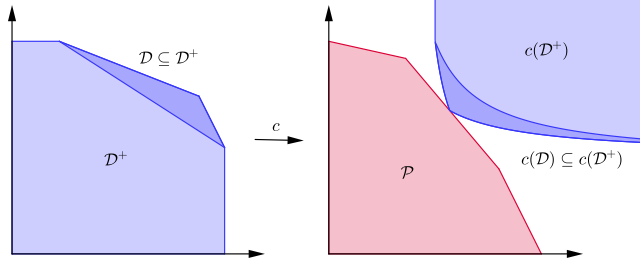Figure 1. The centroid map.

This means the primal optimum is the unique point in the intersection $\mathcal{P} \cap \mathcal{D}^+$. $c(\mathcal{D}^+)$ is convex, so this is a linear feasibility problem over a convex set. We use Plotkin-Smoys-Tardos (PST) for this problem, in a version inspired by [AHK12] which is better suited for this purpose.

The PST algorithm requires an oracle which for a given “query” halfspace $h$ returns a point $x\in c(\mathcal{D}^+)$ such that $\langle h, x\rangle \leq 1$. The closer the oracle returns points to the optimum $x^{opt}$, the faster our algorithm will run. The oracle we suggest depends on a feasible solution to (dual), and its performance improves as the solution is closer to optimum in (dual).

In particular, our oracle depends on a solution $s$ and the points it returns are in a region we call the lens of $s$, $\mathcal{L}_{\delta}$: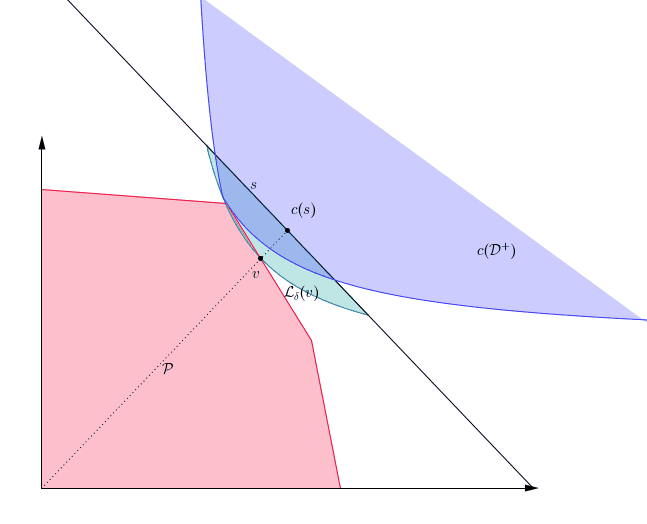Figure 2. The primal polytope $P$, and the lens of a feasible solution.

As the figure illustrates, the lens of $s$ becomes smaller as $s$ improves as a solution. For this reason, we use a restart scheme: First we compute some approximate solution, then we use that approximate solution as the input for the oracle in the next restart. With this approach, we attain the following result:

Theorem 9. Let $\varepsilon \in (0,n(n-1)]$ be an accuracy parameter. There is an algorithm that finds a linear combination of the rows of $A$, $\lambda\in\Delta^m$ such that $g(\lambda)$ is an $(\varepsilon/n)$-approximate solution of (dual) after $\widetilde{O}( n^2/\varepsilon)$ iterations.

#### A potential application: The Yamnitsky-Levin simplices algorithm

The Yamnitsky-Levin algorithm [YL82] is an algorithm for linear feasibility problem. It is very similar to the ellipsoid method:

Input: A matrix $A\in\mathbb{R}^{m\times n}_{\geq 0}$,and a vector $b\in\mathbb{R}$

Output: Either a point $x\in\mathcal{P}$ where $P={x\in \mathbb{R}_{\geq 0} : Ax\leq b }$ or the guarantee that $\mathcal{P}$ has volume $\leq \varepsilon$.

• start with a simplex $\Delta$ covering $\mathcal{P}$.
• while the centroid of $\Delta$, $c$ is not in $\mathcal{P}$
• find a hyperplane separating $c$ from $\mathcal{P}$ (a row of $Ax\leq b$).
• Combine the separating hyperplane with $\Delta$ to find a new simplex $\Delta$ with smaller volume.
• return c

The interested reader can see the details in [YL82]. Observe that if we choose a suitable change of basis, we can map any $(d-1)$ facets of $\Delta$ to the first orthant. The Yamnitsky-Levin algorithm tries to find some hyperplane for the last facet minimizing the simplex volume while covering $\mathcal{P}$.

Recall that this is exactly what (dual) is, except we are only considering the positive constraints. In a way, (dual) is solving the Yamnitsky-Levin problem but with two changes: it considers more than one constraint at the same time, but it can only change one facet of the simplex at a time.

It is possible to replace the Yamnitsky-Levin simplex pivoting step with the algorithm in Theorem 9. However, it is not clear yet how this affects its performance.

We would like to thank Prof. Elias Koutsoupias for starting our motivation in this problem.

### References

[AK08] Baruch Awerbuch and Rohit Khandekar. Stateless distributed gradient descent for positive linear programs. Proceedings of the fourtieth annual ACM symposium on Theory of computing - STOC 08, page 691, 2008

[AO17] Zeyuan Allen-Zhu and Lorenzo Orecchia. Linear coupling: An ultimate unification of gradient and mirror descent. In Christos H. Papadimitriou, editor, 8th Innovations in Theoretical Computer Science Conference, ITCS 2017, January 9-11, 2017, Berkeley, CA, USA, volume 67 of LIPIcs, pages 3:1–3:22. Schloss Dagstuhl - Leibniz-Zentrum für Informatik, 2017.

[AO19] Zeyuan Allen-Zhu and Lorenzo Orecchia. Nearly linear-time packing and covering LP solvers achieving width-independence and 1/epsilon-convergence. Math. Program., 175(1-2):307–353, 2019. doi: 10.1007/s10107-018-1244-x. URL https://doi.org/10.1007/s10107-018-1244-x.

[AHK12] Sanjeev Arora, Elad Hazan, and Satyen Kale. The multiplicative weights update method: a meta-algorithm and applications. Theory Comput., 8(1):121–164, 2012. doi: 10.4086/toc.2012. v008a006. URL https:// doi.org/ 10.4086/ toc.2012.v008a006.

[BF11] Dimitris Bertsimas, Vivek F. Farias, and Nikolaos Trichakis. The price of fairness. Oper. Res., 59 (1):17–31, 2011. doi: 10.1287/opre.1100.0865. URL https://doi.org/10.1287/opre.1100.0865.

[LKCS10] Tian Lan, David T. H. Kao, Mung Chiang, and Ashutosh Sabharwal. An axiomatic theory of fairness in network resource allocation. In INFOCOM 2010. 29th IEEE International Conference on Computer Communications, Joint Conference of the IEEE Computer and Communications Societies, 15-19 March 2010, San Diego, CA, USA, pages 1343–1351. IEEE, 2010. doi: 10.1109/ INFCOM.2010.5461911. URL https://doi.org/10.1109/INFCOM.2010.5461911.

[BNOT14] Amir Beck, Angelia Nedic, Asuman E. Ozdaglar, and Marc Teboulle. An $O(1/k)$ gradient method for network resource allocation problems. IEEE Trans. Control. Netw. Syst., 1(1):64–73, 2014. doi: 10.1109/TCNS.2014.2309751. URL https://doi.org/10.1109/TCNS.2014.2309751.

[MSZ16] Jelena Marašević, Clifford Stein, and Gil Zussman. A fast distributed stateless algorithm for alpha-fair packing problems. In Ioannis Chatzigiannakis, Michael Mitzenmacher, Yuval Rabani, and Davide Sangiorgi, editors, 43rd International Colloquium on Automata, Languages, and Programming, ICALP 2016, July 11-15, 2016, Rome, Italy, volume 55 of LIPIcs, pages 54:1–54:15. Schloss Dagstuhl - Leibniz-Zentrum für Informatik, 2016. doi: 10.4230/LIPIcs.ICALP.2016.54. URL https://doi.org/10.4230/LIPIcs.ICALP.2016.54.

[DFO20] Jelena Diakonikolas, Maryam Fazel, and Lorenzo Orecchia. Fair packing and covering on a relative scale. SIAM J. Optim., 30(4):3284–3314, 2020. doi: 10.1137/19M1288516. URL https://doi.org/10.1137/19M1288516.

[N50] John F. Nash. The bargaining problem. Econometrica, 18(2):155–162, 1950. ISSN 00129682, 14680262. URL http://www.jstor.org/stable/1907266.

[YL82] Boris Yamnitsky and Leonid A. Levin. An old linear programming algorithm runs in polynomial time. In 23rd Annual Symposium on Foundations of Computer Science, Chicago, Illinois, USA, 3-5 November 1982, pages 327–328. IEEE Computer Society, 1982.

]]>
Francisco Criado, David Martínez-Rubio
Simple steps are all you need2021-10-09T07:00:00+02:002021-10-09T07:00:00+02:00http://www.pokutta.com/blog/research/2021/10/09/self-concordant-abstractTL;DR: This is an informal summary of our recent paper, to appear in NeurIPS’21, Simple steps are all you need: Frank-Wolfe and generalized self-concordant functions by Alejandro Carderera, Mathieu Besançon, and Sebastian Pokutta, where we present a monotonous version of the Frank-Wolfe algorithm, which together with the simple step size $\gamma_t = 2/(2+t)$ achieves a $\mathcal{O}\left( 1/t \right)$ convergence in primal gap and Frank-Wolfe gap when minimizing Generalized Self-Concordant (GSC) functions over compact convex sets.

Written by Alejandro Carderera.

## What is the paper about and why you might care

Consider a problem of the sort: \tag{minProblem} \begin{align} \label{eq:minimizationProblem} \min\limits_{x \in \mathcal{X}} f(x), \end{align} where $\mathcal{X}$ is a compact convex set and $f(x)$ is a generalized self-concordant (GSC) function. This class of functions, which can informally be defined as those whose third derivative is bounded by their second derivative, have played an important role in the development of polynomial time algorithms for optimization, and also happen to appear in many machine learning problems. For example, the objective function encountered in logistic regression, or in marginal inference with concave maximization [KLS], belong to this family of functions.

As in previous posts, our focus is on Frank-Wolfe or Conditional Gradient algorithms, and we assume that solving an LP over $\mathcal{X}$ is easy, but projecting onto $\mathcal{X}$ is hard, additionally, we assume access to first-order and zeroth-order information about the function. Existing algorithms for this class of functions require access to second-order information, or local smoothness estimates, to achieve a $\mathcal{O}\left( 1/t \right)$ rate of convergence in primal gap [DSSS]. With the Monotonous Frank-Wolfe (M-FW) algorithm we require neither of these, achieving a $\mathcal{O}\left( 1/t \right)$ rate both in primal gap and Frank-Wolfe gap with a simple six-line algorithm that only requires access to a domain oracle, which is simply an oracle that checks if $x \in \mathrm{dom} f$. This extra oracle has to be used if one is to avoid assuming access to second-order information, and is also implicitly used by the existing algorithms that compute local estimates of the smoothness. The proof of convergence for both primal gap and Frank-Wolfe gap are simple and easy to follow, which add to the appeal of the algorithm.

Additionally, we also show improved rates of convergence with a backtracking line search [PNAM] (that locally estimates the smoothness) when the optimum is contained in the interior of $\mathcal{X} \cap \mathrm{dom} f$, when $\mathcal{X}$ is uniformly convex or when $\mathcal{X}$ is a polytope. The contributions are summarized in the table below.## The Monotonous Frank-Wolfe (M-FW) algorithm

Many convergence proofs in optimization make use of the smoothness inequality to bound the progress that an algorithm makes per iteration when moving from $x_{t}$ to $x_{t} + \gamma_t (v_{t} - x_{t})$. For smooth functions this inequality holds globally for all $x_{t}$ and $x_{t} + \gamma_t (v_{t} - x_{t})$. For GSC functions we also have a smoothness-like inequality with which we can bound progress. The problem is that this inequality only holds locally around $x_t$, and if one wants to test if the smoothness-like inequality is valid between $x_t$ and $x_{t} + \gamma_t (v_{t} - x_{t})$ we need to have knowledge of $\nabla^2 f(x_t)$, and know several parameters of the function. Several of the algorithms presented in [DSSS] utilize this approach, in order to compute a step size $\gamma_t$ such that the smoothness-like inequality holds between $x_t$ and $x_{t} + \gamma_t (v_{t} - x_{t})$. Alternatively, one can use the backtracking line search of [PNAM] to find a $\gamma_t$ and a smoothness estimate such that a local smoothness inequality holds between $x_t$ and $x_{t} + \gamma_t (v_{t} - x_{t})$.

We take a different approach to prove a convergence bound, which we review after describing our algorithm. The Monotonous Frank-Wolfe (M-FW) algorithm below is a rather simple, but powerful modification of the standard Frank-Wolfe algorithm, with the only difference that before taking a step, we verify if $x_t +\gamma_t \left( v_t - x_t\right) \in \mathrm{dom} f$, and if so, we check whether moving to the next iterate provides primal progress. Note, that the open-loop step size rule $2/(2+t)$ does not guarantee monotonous primal progress for the vanilla Frank-Wolfe algorithm in general. If either of these two checks fails, we simply do not move: the algorithm sets $x_t = x_{t+1}$. Note that if this is the case we do not need to compute a gradient or an LP call at iteration $t+1$, as we can simply reuse $v_t$.

Monotonous Frank-Wolfe (M-FW) algorithm
Input: Initial point $x_0 \in \mathcal{X}$
Output: Point $x_{T+1} \in \mathcal{X}$
For $t = 1, \dots, T$ do:
$$\quad v_t \leftarrow \mathrm{argmin}_{v \in \mathcal{X}}\left\langle \nabla f(x_t),v \right\rangle$$
$$\quad \gamma_t \leftarrow 2/(2+t)$$
$$\quad x_{t+1} \leftarrow x_t +\gamma_t \left( v_t - x_t\right)$$
$$\quad \text{if } x_{t+1} \notin \mathrm{dom} f \text{ or } f(x_{t+1}) > f(x_t) \text{ then}$$
$$\quad \quad x_{t + 1} \leftarrow x_t$$
End For

The simple structure of the algorithm above allows us to prove a $\mathcal{O}(1/t)$ convergence bound in primal gap and Frank-Wolfe gap. To do this we use an inequality that holds if $d(x_t +\gamma_t \left( v_t - x_t\right), x_t) \leq 1/2$, where $d(x,y)$ is a distance function that depends on the structure of the GSC function. Namely the inequality that we use is: \begin{align} f(x_t +\gamma_t \left( v_t - x_t\right)) - f(x^*) \leq (f(x_t)-f(x^*))(1-\gamma_t) + \gamma_t L_{f,x_0}D^2 \omega(1/2), \end{align} where $D$ is the diameter of $\mathcal{X}$, and $L_{f,x_0}$ and $\omega(1/2)$ are constants that depend on the function, and the starting point (which we do not need to know). If we were to have knowledge of $\nabla^2 f(x_t)$ we could compute the value of $\gamma_t$ that allows us to ensure that $d(x_t +\gamma_t \left( v_t - x_t\right), x_t) \leq 1/2$, however we purposefully do not want to use second order information!

We briefly (and informally) describe how we prove this convergence bound for the primal gap: As the iterates make monotonous progress, and the step size $\gamma_t = 2/(2+t)$ in our scheme decreases continously, there is an iteration $T$, which depends on the function, after which the smoothness-like inequality holds for all $t \geq T$ between $x_t$ and $x_t +\gamma_t \left( v_t - x_t\right)\in \mathrm{dom} f$, i.e. we guarantee that $d(x_t +\gamma_t \left( v_t - x_t\right), x_t) \leq 1/2$ for $t \geq T$ (without the need to know any parameters). However, note that in order to take a non-zero step size we also need to ensure that $f(x_{t+1}) < f(x_t)$. We complete the convergence proof using induction, that is, the assumption that $f(x_t) - f(x^\esx) \leq C(T+1)/(t+1)$ where $C$ is a constant, and the following subtlety – the smoothness-like inequality will only guarantee progress (i.e. $f(x_{t+1}) < f(x_t)$) at iteration $t$ if $\gamma_t$ is smaller than the primal gap at iteration $t$ multiplied by a factor. We can see this by going to the inequality above, and seeing that we will be able to guarantee that $f(x_{t+1}) < f(x_t)$ if: \begin{align} \gamma_t(f(x_t) - f(x^*)) - \gamma_t^2L_{f,x_0}D^2 \omega(1/2) <0, \end{align} If this is true, we can guarantee that we set $x_{t+1} = x_t +\gamma_t \left( v_t - x_t\right)$ and we can bound the progress using the smoothness-like inequality. Using the aforementioned fact and our induction hypothesis that $$f(x_t) - f(x^\esx) \leq C(T+1)/(t+1)$$, we prove that claim $f(x_{t+1}) - f(x^\esx) \leq C(T+1)/(t+2)$. Assume however that this is not the case and that the following inequality is true: \begin{align} \gamma_t(f(x_t) - f(x^*)) - \gamma_t^2L_{f,x_0}D^2 \omega(1/2) \geq 0. \end{align} Reordering the previous expression, we have that $f(x_t) - f(x^*) \leq \gamma_t L_{f,x_0}D^2 \omega(1/2)$, with $\gamma_t = 2/(2+t)$. It turns out that $\gamma_t L_{f,x_0}D^2 \omega(1/2) \leq C(T+1)/(t+2)$, so there is nothing left to prove, and we do not even need the induction hypothesis, as for this case the claim is automatically true for $t+1$. The proof of convergence in Frank-Wolfe gap proceeds similarly. See Theorem 2.5 and Theorem A.2 in the paper for the full details.

### Complexity Analysis

As each iteration of the algorithm computes at most one first-order oracle call, one zeroth-order oracle call, one LP call, and one domain oracle call, we can bound the number of oracle calls needed to achieve an $\epsilon$ tolerance in primal gap (or Frank-Wolfe gap) directly using the iteration-complexity bound of $\mathcal{O}(1/\epsilon)$.

Note also that we can also implement the M-FW algorithm using a halving strategy for the step size, instead of the $2/(2+t)$ step size. This strategy helps deal with the case in which a large number of consecutive step sizes $\gamma_t$ are rejected either because $x_t + \gamma_t(v_t - x_t) \notin \mathrm{dom} f$ or $f(x_t) < f(x_t + \gamma_t(v_t - x_t))$. The strategy consists of halving the step size if we encounter any of these two cases. This results in a step size that is at most a factor of 2 smaller than the one that would have been accepted with the original strategy. However, the number of zeroth-order or domain oracles that would be needed to find this step size that satisfies the desired properties is logarithmic when compared to the number needed for the $2/(2+t)$ variant. The convergence properties established throughout the paper for the M-FW also hold for the variant with the halving strategy; with the only difference being that we lose a small constant factor in the convergence rate.

### Computational Experiments

We compare the performance of the M-FW algorithm with that of other projection free algorithms which apply to the GSC setting. That is, we compare to the B-FW and the GSC-FW algorithms of [DSSS], the non-monotonous standard FW algorithm, for which there are no formal convergence guarantees for this class of problems, and the B-AFW algorithm. Note that the B-AFW is simply the AFW algorithm with the backtracking strategy of [PNAM], for which we also provide convergence guarantees in some special cases in the paper for GSC functions.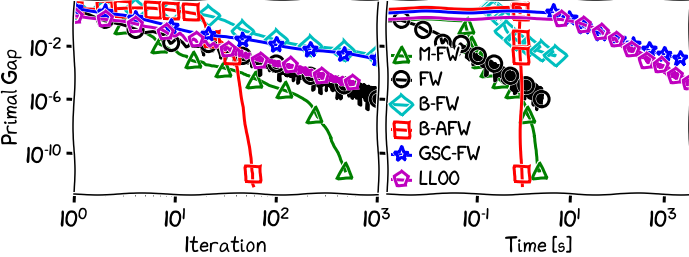Figure 1. Portfolio Optimization.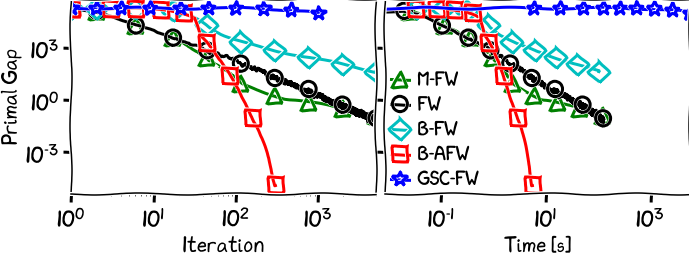Figure 2. Signal recovery with KL divergence.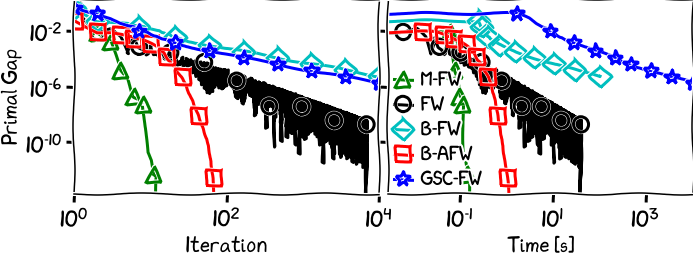Figure 3. Logistic regression over $\ell_1$ unit ball.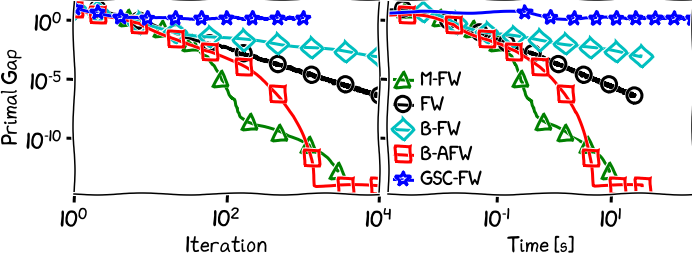Figure 4. Logistic regression over the Birkhoff polytope.

### References

[KLS] Krishnan, R. G., Lacoste-Julien, S., and Sontag, D. Barrier Frank-Wolfe for Marginal Inference. In Proceedings of the 28th Conference in Neural Information Processing Systems. PMLR, 2015. pdf

[DSSS] Dvurechensky, P., Safin, K., Shtern, S., and Staudigl, M. Generalized self-concordant analysis of Frank-Wolfe algorithms. arXiv preprint arXiv:2010.01009, 2020b. pdf

[PNAM] Pedregosa, F., & Negiar, G. & Askari, A. & Jaggi, M. (2020). Linearly Convergent Frank-Wolfe with Backtracking Line-Search. In Proceedings of the 23rd International Conference on Artificial Intelligence and Statistics. pdf

]]>
Alejandro Carderera
New(!!) NeurIPS 2021 competition: Machine Learning for Discrete Optimization (ML4CO)2021-06-18T07:00:00+02:002021-06-18T07:00:00+02:00http://www.pokutta.com/blog/news/2021/06/18/NeurIPS-ML4CO-competitionTL;DR: This year at NeurIPS 2021 there is brand new competition: improving integer programming solvers by machine learning. The learning problems are quite different than your usual suspects requiring non-standard learning approaches.

So you are tired of classifying cats and dogs? You are done with Kaggle competitions? How about trying something new? This year at NeurIPS there will be a new competition „Machine Learning for Discrete Optimization (ML4CO)“ which is about improving Integer Programming Solvers by means of Machine Learning.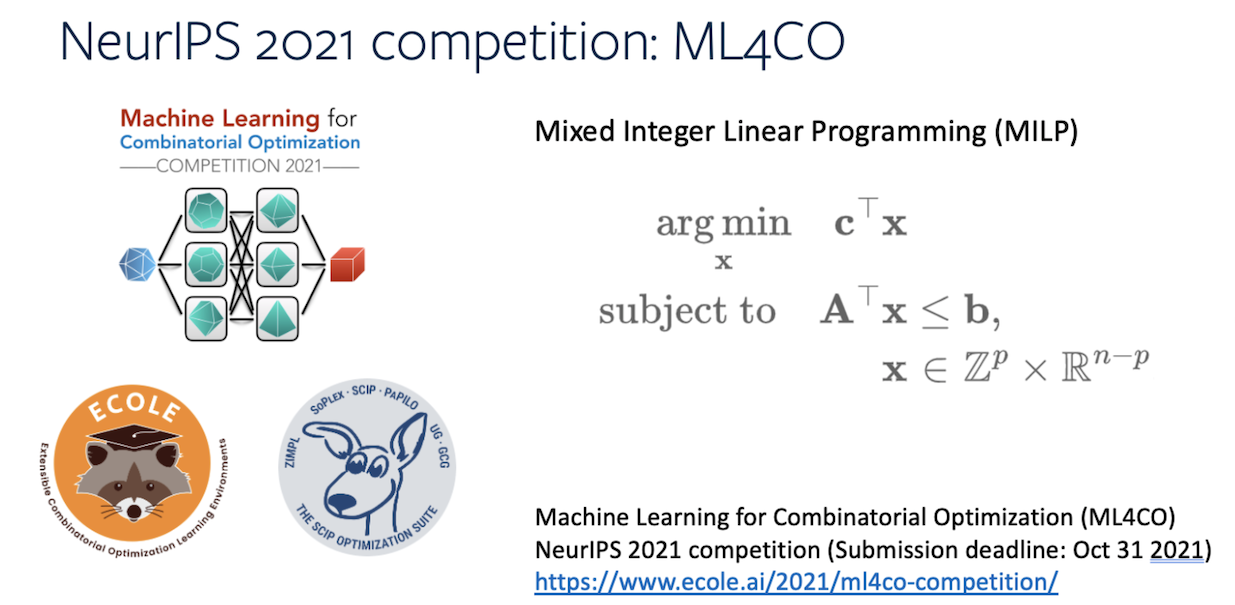In contrast to many other learning tasks the associated learning problems have a couple of characteristics that make them especially hard:

1. Sampling and data acquisition is usually quite expensive and noisy
2. There are strong interactions between decisions
3. There are lots of long-range dependencies

So if you want to try something different, this might be a good chance. The (semi-)official announcement roughly reads as follows:

The Machine Learning for Combinatorial Optimization (ML4CO) NeurIPS 2021 competition aims at improving state-of-the-art combinatorial optimization solvers by replacing / integrating key heuristic components with machine learning models. The competition’s main scientific question is the following: is machine learning a viable option for improving traditional combinatorial optimization solvers on specific problem distributions, when historical data is available?

The webpage of the competition with all necessary information is

https://www.ecole.ai/2021/ml4co-competition

and the preregistration form is

https://forms.gle/pv6aaXxZ9iGYVCtj9

The ML4CO organizers

There will be three tasks that one can compete in this year: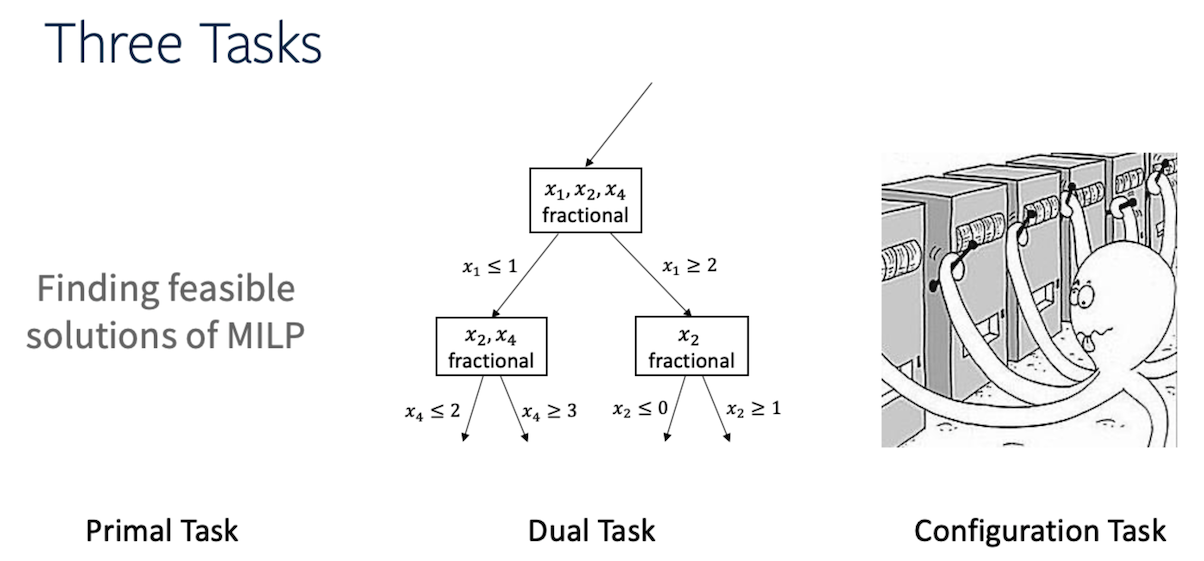The first one is really about primal solutions: producing new feasible solutions with good objective function values fast in order to minimize the so-called primal integral.

The second task is about closing the dual gap: learning to select branching variables. This can have many positive effects in the solution process and the aggregate measure that is considered here is the dual integral.

Finally, the third task is more of a traditional configuration learning task. Integer Programming solvers’ performance heavily depends on the chosen parameters. The task here is to learn a good set of parameters for a given problem instance and the considered metric is the primal-dual integral.

This competition is gonna be lit 🔥 and the whole team is super excited to see what y’all come up with!

]]>
Sebastian Pokutta
Learning to Schedule Heuristics in Branch and Bound2021-05-06T01:00:00+02:002021-05-06T01:00:00+02:00http://www.pokutta.com/blog/research/2021/05/06/learningHeuristicsTL;DR: This is an informal discussion of our recent paper Learning to Schedule Heuristics in Branch and Bound by Antonia Chmiela, Elias Khalil, Ambros Gleixner, Andrea Lodi, and Sebastian Pokutta. In this paper, we propose the first data-driven framework for scheduling heuristics in a MIP solver. By learning from data describing the performance of primal heuristics, we obtain a problem-specific schedule of heuristics that collectively find many solutions at minimal cost. We provide a formal description of the problem and propose an efficient algorithm for computing such a schedule.

Written by Antonia Chmiela.

### Motivation

Primal heuristics play a crucial role in exact solvers for Mixed Integer Programming (MIP). For instance, Berthold  showed that the primal bound improved on average by around 80% when primal heuristics were used. While solvers are guaranteed to find optimal solutions given sufficient time, real-world applications typically require finding good solutions early on in the search to enable fast decision-making. Even though much of MIP research focuses on designing effective heuristics, the question of how to manage multiple MIP heuristics in a solver has not received equal attention.

Generally, a solver has a variety of primal heuristics implemented, where each class exploits a different idea to find good solutions. During Branch and Bound (B&B), these heuristics are executed successively at each node of the search tree, and improved solutions are reported back to the solver if found. Since most heuristics can be very costly, it is necessary to be strategic about the order in which the heuristics are executed and the number of iterations allocated to each, with the ultimate goal of obtaining good primal performance overall. Such decisions are often made by following hard-coded rules derived from testing on broad benchmark test sets. While these static settings yield good performance on average, their performance can be far from optimal when considering specific families of instances.

In this paper, we propose a data-driven approach to systematically improve the use of primal heuristics in B&B. By learning from data about the duration and success of every heuristic call for a set of training instances, we construct a schedule of heuristics deciding when and for how long a certain heuristic should be executed to obtain good primal solutions early on. As a result, we are able to significantly improve the use of primal heuristics.

### Contributions

Our main contributions can be summarized as follows:

1. We formalize the learning task of finding an effective, cost-efficient heuristic schedule on a training dataset as a Mixed Integer Quadratic Program;
2. We propose an efficient heuristic for solving the training (scheduling) problem and a scalable data collection strategy;
3. We perform extensive computational experiments on two classes of challenging instances and demonstrate the benefits of our approach.

### Obtaining a Heuristic Schedule

We consider the following practically relevant setting. We are given a set of heuristics $\mathcal{H}$ and a homogeneous set of training instances $\mathcal{X}$ from the same problem class we are interested to solve in practice. In a data collection phase, we are allowed to execute the B&B algorithm on the training instances, observing how each heuristic performs at each node of each search tree. At a high level, our goal is then to leverage this data to obtain a schedule of heuristics that minimizes a primal performance metric.

A heuristic schedule controls two important aspects: The order in which a set of applicable heuristics $\mathcal{H}$ is executed and the maximal duration of each heuristic run. To find primal solutions, the solver executes a heuristic loop that iterates over the heuristics in decreasing priority. The loop is terminated if a heuristic finds a new incumbent solution. As such, an ordering that prioritizes effective heuristics can lead to time savings without sacrificing primal performance. Furthermore, solvers use working limits to control the computational effort spent on heuristics. By allowing a heuristic to be more expensive, i.e., increase the overall running time, we also increase the likelihood of finding an integer feasible solution. Hence, a heuristic schedule is defined as follows. For a heuristic $h \in$ $\mathcal{H}$, let $\tau \in \mathbb{R}_{>0}$ denote $h$’s time budget. Then, we are interested in finding a schedule $S$ defined by

$S := \langle (h_1, \tau_1), \dots, (h_k, \tau_k) \rangle, h_i \in \mathcal{H}.$

We also refer to $\tau_i$ as the maximal number of iterations that is allocated to a $h_i$ in schedule $S$.

Furthermore, let us denote by $\mathcal{N}_{\mathcal{X}}$ the collection of search tree nodes that appear when solving the instances in $\mathcal{X}$ with B&B. Recall that our objective is to optimize the use of primal heuristics such that we find feasible solutions fast. To achieve this, we learn from the data and construct a schedule $S$ that finds feasible solutions for a large fraction of the nodes in $\mathcal{N}_X$, while also minimizing the number of iterations spent by schedule $S$. Hence, the heuristic scheduling problem we consider is given by

$\begin{equation} \tag{P_{\mathcal{S}}} \underset{S \in \mathcal{S}}{\text{min}} \sum_{N \in \mathcal{N}_{\mathcal{X}}} T(S,N) \;\text{ s.t. }\; |\mathcal{N}_S| \geq \alpha |\mathcal{N}_{\mathcal{X}}|. \end{equation}$

Here $T(S,N)$ denotes the number of iterations schedule $S$ needs to solve node $N$ and $N_S$ is the set of nodes at which schedule $S$ is successful in finding a solution. The parameter $\alpha \in [0,1]$ denotes a minimum fraction of nodes at which we want the schedule to find a solution. Problem ($P_{\mathcal{S}}$) can be formulated as a Mixed-Integer Quadratic Program (MIQP).

To find such a schedule, we need to know the number of iteration it takes heuristic $h$ to solve node $N$ for all heuristics in $\mathcal{H}$ and nodes $\mathcal{N}_{\mathcal{X}}$. Hence, when collecting data for the instances in the training set $\mathcal{X}$, we track for every B&B node $N$ at which a heuristic $h$ was called, the number of iterations $\tau^h_N$ it took $h$ to find a feasible solution. We propose an efficient data collection framework that uses a specially crafted version of a MIP solver for collecting multiple reward signals for the execution of multiple heuristics per single MIP evaluation during the training phase. As a result, we obtain a large amount of data points that scales with the running time of the MIP solves.

Unfortunately, the problem ($P_{\mathcal{S}}$) is $\mathcal{NP}$-hard and too expensive to solve in practice, so we direct our attention towards designing an efficient heuristic algorithm. The approach we propose follows a greedy tactic and the basic idea can be summarized as follows: A schedule $G$ is built by successively adding the action $(h,\tau)$ to $G$ that maximizes the ratio of the marginal increase in the number of instances solved to the cost (w.r.t. a cost fuction $c$) of including $(h,\tau)$. In other words, we start with an empty schedule $G_0 = \langle \rangle$ and successively add actions more detailed description as well as the pseudo-code can be found in our paper.

\begin{equation*} \begin{aligned} g_j = \underset{(h,\tau)}{\text{argmax}} \frac{|\{ N \in \mathcal{N}_{\mathcal{X}}\setminus \mathcal{N}_{\mathcal{G}_{j-1}} \mid \tau_N^h \leq \tau\}|}{c_{j-1}(h,\tau)}, \end{aligned} \end{equation*}

until either all nodes in $\mathcal{N}_{\mathcal{X}}$ are solved by $G_j$ or all heuristics are already contained in the schedule. A more detailed description as well as the pseudo-code can be found in our paper. Our code can be found here.

### Sneak Peak at the Results

A comprehensive experimental evaluation shows that our approach consistently learns heuristic schedules with better primal performance than the default settings of the state-of-the-art academic MIP solver SCIP. For instance, we are able to reduce the average primal integral by up to 49% on two classes of challenging instances – namely the Generalized Independent Set Problem (GISP)  and Fixed-Charge Multicommodity Network Flow Problem (FCMNF) . A brief comparison of the average primal integral over time is shown in the following figure.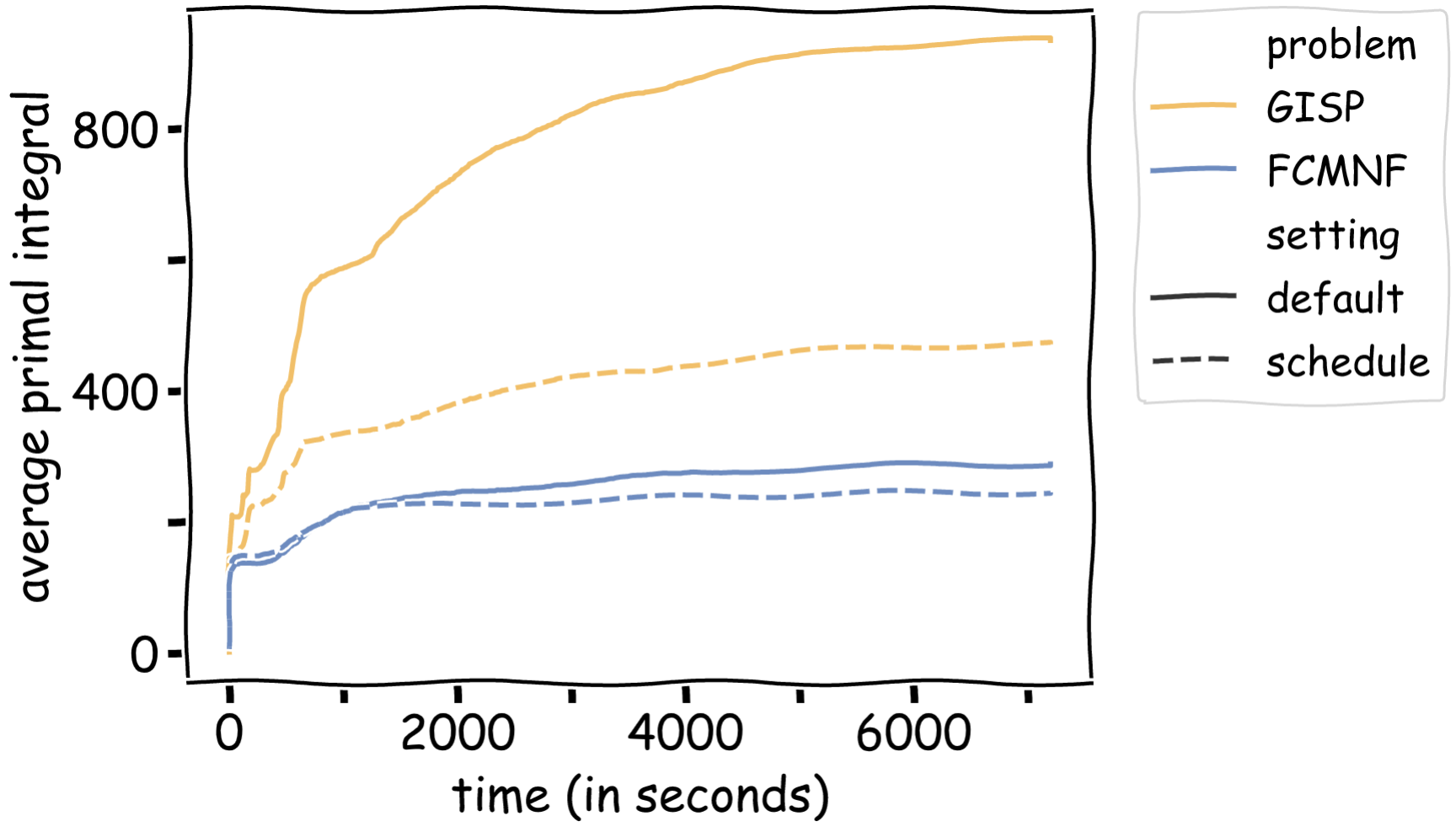The average primal integral is not the only performance metric for which we observed a significant improvement. On average, the instances solved with the schedule terminated with a smaller primal-dual gap, a better primal bound (for instances that hit the time limit) and overall found more solutions during the solving process.

### References

 Timo Berthold. Measuring the impact of primal heuristics. Operations Research Letters 41.6 (2013): 611-614. [pdf]

 Marco Colombi, Renata Mansini, Martin Savelsbergh. The generalized independent set problem: Polyhedral analysis and solution approaches. European Journal of Operational Research 260.1 (2017): 41-55. [pdf]

 Lluís-Miquel Munguía, Shabbir Ahmed, David A. Bader, George L. Nemhauser, Vikas Goel, Yufen Shao. A parallel local search framework for the Fixed-Charge Multicommodity Network Flow problem. Computers & Operation Research 77 (2017): 44-57. [pdf]

]]>
Antonia Chmiela
FrankWolfe.jl: A high-performance and flexible toolbox for Conditional Gradients2021-04-20T07:00:00+02:002021-04-20T07:00:00+02:00http://www.pokutta.com/blog/research/2021/04/20/FrankWolfejlTL;DR: We present $\texttt{FrankWolfe.jl}$, an open-source implementation in Julia of several popular Frank-Wolfe and Conditional Gradients variants for first-order constrained optimization. The package is designed with flexibility and high-performance in mind, allowing for easy extension and relying on few assumptions regarding the user-provided functions. It supports Julia’s unique multiple dispatch feature, and interfaces smoothly with generic linear optimization formulations using $\texttt{MathOptInterface.jl}$.

Written by Alejandro Carderera and Mathieu Besançon.

## What does the package do?

The $\texttt{FrankWolfe.jl}$ Julia package aims at solving problems of the form: \tag{minProblem} \begin{align} \label{eq:minimizationProblem} \min\limits_{\mathbf{x} \in \mathcal{C}} f(\mathbf{x}), \end{align} where $\mathcal{C} \subseteq \mathbb{R}^d$ is a convex compact set and $f(\mathbf{x})$ is a differentiable function, through the use of Frank-Wolfe [FW] (also known as Conditional Gradient [LP]) algorithm variants. The two main ingredients that the package uses to solve this problem are:

1. A First-Order Oracle (FOO): Given $\mathbf{x} \in \mathcal{C}$, the oracle returns $\nabla f(\mathbf{x})$.
2. A Linear Minimization Oracle (LMO): Given $\mathbf{x}\in \mathbb{R}^d$, the oracle returns $\mathbf{v} \in \operatorname{argmin}_{\mathbf{x} \in \mathcal{C}} \langle \mathbf{d}, \mathbf{x}\rangle$.

This bypasses the need to use projection oracles onto $\mathcal{C}$, which can be extremely advantageous as solving an LP over $\mathcal{C}$ can be much cheaper than solving a quadratic (projection) problem over the same set. Such is the case for the nuclear norm ball, as solving an LP over this convex set simply requires computing the left and right singular vectors associated with the largest singular value, whereas projecting onto this feasible region requires computing a full SVD decomposition. See [CP] for more examples and for more background information on the package see also our software overview of the package [BCP].

## How do I get started

In a Julia session, type ] to switch to package mode:

julia> ]
(@v1.6) pkg>


See the Julia documentation for more examples and advanced usage of the package manager. You can then add the package with the add command:

(@v1.6) pkg> add https://github.com/ZIB-IOL/FrankWolfe.jl


Soon it will also be directly available through the package manager.

## And why should you care?

Although the Frank-Wolfe algorithm and its variants have been studied for more than half a decade and have gained a lot of attention due to their favorable theoretical and computational properties, no de-facto implementation exists. The goal of the package is to become a reference open-source implementation for practitioners in need of a flexible and efficient first-order method and for researchers developing and comparing new approaches on similar classes of problems.

## Algorithm variants included in the package

We summarize below the central ideas of the variants implemented in the package and highlight in Table 1 key properties that can drive the choice of a variant on a given use case. More information about the variants can be found in the references provided. We mention briefly that most variants also work for the nonconvex case, providing some locally optimal solution in this case.

Standard Frank-Wolfe. The simplest Frank-Wolfe variant is included in the package. It has the lowest memory requirements out of all the variants, as in its simplest form only requires keeping track of the current iterate. As such it is suited for extremely large problems. However, in certain cases, this comes at the cost of speed of convergence in terms of iteration count, when compared to other variants. As an example, when minimizing a strongly convex and smooth function over a polytope this algorithm might converge sublinearly, whereas the three variants that will be presented next converge linearly.

Away-step Frank-Wolfe. One of the most popular Frank-Wolfe variants is the Away-step Frank-Wolfe (AFW) algorithm [GM, LJ]. While the standard FW algorithm can only move towards extreme points of $\mathcal{C}$, the AFW can move away from some extreme points of $\mathcal{C}$, hence the name of the algorithm. To be more specific, the AFW algorithm moves away from vertices in its active set at iteration $t$, denoted by $\mathcal{S}_t$, which contains the set of vertices $\mathbf{v}_k$ for $k<t$ that allow us to recover the current iterate as a convex combination. This algorithm expands the range of directions that the FW algorithm can move along, at the expense of having to explicitly maintain the current iterate as a convex decomposition of extreme points.

Lazifying Frank-Wolfe variants. One running assumption for the two previous variants is that calling the LMO is cheap. There are many applications where calling the LMO in absolute terms is costly (but is cheap in relative terms when compared to performing a projection). In such cases, one can attempt to lazify FW algorithms, to avoid having to compute $\operatorname{argmin}_{\mathbf{v}\in\mathcal{C}}\left\langle\nabla f(\mathbf{x}_t),\mathbf{v} \right\rangle$ by calling the LMO, settling for solutions that guarantee enough progress [BPZ]. This allows us to substitute the LMO by a Weak Separation Oracle while maintaining essentially the same convergence rates. In practice, these algorithms search for appropriate vertices among the vertices in a cache, or the vertices in the active set $\mathcal{S}_t$, and can be much faster in wall-clock time. In the package, both AFW and FW have lazy variants while the BCG algorithm is lazified by design.

Blended Conditional Gradients. The FW and AFW algorithms, and their lazy variants share one feature: they attempt to make primal progress over a reduced set of vertices. The AFW algorithm does this through away steps (which do not increase the cardinality of the active set), and the lazy variants do this through the use of previously exploited vertices. A third strategy that one can follow is to explicitly blend Frank-Wolfe steps with gradient descent steps over the convex hull of the active set (note that this can be done without requiring a projection oracle over $\mathcal{C}$, thus making the algorithm projection-free). This results in the Blended Conditional Gradient (BCG) algorithm [BPTW], which attempts to make as much progress as possible over the convex hull of the current active set $\mathcal{S}_t$ until it automatically detects that in order to further make further progress it requires additional calls to the LMO and new atoms.

Stochastic Frank-Wolfe. In many problem instances, evaluating the FOO at a given point is prohibitively expensive. In such cases, one usually has access to a Stochastic First-Order Oracle (SFOO), from which one can build a gradient estimator. This idea, which has powered much of the success of deep learning, can also be applied to the Frank-Wolfe algorithm [HL], resulting in the Stochastic Frank-Wolfe (SFW) algorithm and its variants.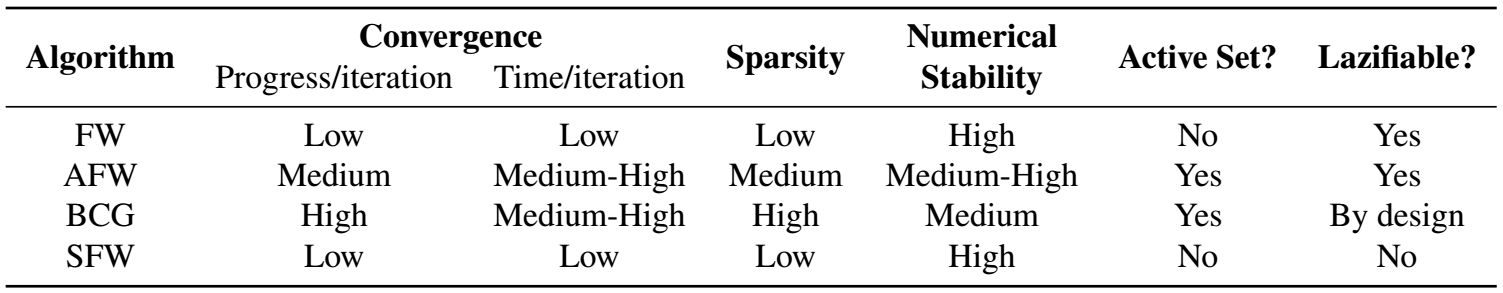Table 1. Schematic comparison of the different algorithmic variants.

## Package design characteristics

Unlike disciplined convex frameworks or algebraic modeling languages such as $\texttt{Convex.jl}$ or $\texttt{JuMP.jl}$, our framework allows for arbitrary Julia functions defined outside of a Domain-Specific Language. Users can provide their gradient implementation or leverage one of the many automatic differentiation packages available in Julia.

One central design principle of $\texttt{FrankWolfe.jl}$ is to rely on few assumptions regarding the user-provided functions, the atoms returned by the LMO, and their implementation. The package works for instance out of the box when the LMO returns Julia subtypes of AbstractArray, representing finite-dimensional vectors, matrices or higher-order arrays.

Another design principle has been to favor in-place operations and reduce memory allocations when possible, since these can become expensive when repeated at all iterations. This is reflected in the memory emphasis mode (the default mode for all algorithms), where as many computations as possible are performed in-place as well as in the gradient interface, where the gradient function is provided with a variable to write into rather than reallocating every time a gradient is computed. The performance difference can be quite pronounced for problems in large dimensions, for example passing a gradient of size 7.5GB on a state-of-the-art machine is about 8 times slower than an in-place update.

Finally, default parameters are chosen to make all algorithms as robust as possible out of the box, while allowing extension and fine tuning for advanced users. For example, the default step size strategy for all (but the stochastic variant) is the adaptive step size rule of [PNAM], which in computations not only usually outperforms both line search and the short step rule by dynamically estimating the Lipschitz constant but also overcomes several issues with the limited additive accuracy of traditional line search rules. Similarly, the BCG variant automatically upgrades the numerical precision for certain subroutines if numerical instabilities are detected.

### Linear minimization oracle interface

One key step of FW algorithms is the linear minimization step which, given first-order information at the current iterate, returns an extreme point of the feasible region that minimizes the linear approximation of the function. It is defined in $\texttt{FrankWolfe.jl}$ using a single function:

function compute_extreme_point(lmo::LMO, direction::D; kwargs...)::V
# ...
end


The first argument $\texttt{lmo}$ represents the linear minimization oracle for the specific problem. It encodes the feasible region $\mathcal{C}$, but also some algorithmic parameters or state. This is especially useful for the lazified FW variants, as in these cases the LMO types can take advantage of caching, by storing the extreme vertices that have been computed in previous iterations and then looking up vertices from the cache before computing a new one.

The package implements LMOs for commonly encountered feasible regions including $L_p$-norm balls, $K$-sparse polytopes, the Birkhoff polytope, and the nuclear norm ball for matrix spaces, leveraging known closed forms of extreme points. The multiple dispatch mechanism allows for different implementations of a single LMO with multiple direction types. The type $\texttt{V}$ used to represent the computed vertex is also specialized to leverage the properties of extreme vertices of the feasible region. For instance, although the Birkhoff polytope is the convex hull of all doubly stochastic matrices of a given dimension, its extreme vertices are permutation matrices that are much sparser in nature. We also leverage sparsity outside of the traditional sense of nonzero entries. When the feasible region is the nuclear norm ball in $\mathbb{R}^{N\times M}$, the vertices are rank-one matrices. Even though these vertices are dense, they can be represented as the outer product of two vectors and thus be stored with $\mathcal{O}(N+M)$ entries instead of $\mathcal{O}(N\times M)$ for the equivalent dense matrix representation. The Julia abstract matrix representation allows the user and the library to interact with these rank-one matrices with the same API as standard dense and sparse matrices.

In some cases, users may want to define a custom feasible region that does not admit a closed-form linear minimization solution. We implement a generic LMO based on $\texttt{MathOptInterface.jl}$, thus allowing users on the one hand to select any off-the-shelf LP, MILP, or conic solver suitable for their problem, and on the other hand to formulate the constraints of the feasible domain using the $\texttt{JuMP.jl}$ or $\texttt{Convex.jl}$ DSL. Furthermore, the interface is naturally extensible by users who can define their own LMO and implement the corresponding $\texttt{compute_extreme_point}$ method.

### Numeric type genericity

The package was designed from the start to be generic over both the used numeric types and data structures. Numeric type genericity allows running the algorithms in extended fixed or arbitrary precision, e.g., the package works out-of-the-box with $\texttt{Double64}$ and $\texttt{BigFloat}$ types. Extended precision is essential for high-dimensional problems where the condition number of computed gradients etc., become too high. For some well-conditioned problems, reduced precision is sometimes sufficient to achieve the desired tolerance. Furthermore, it opens the possibility of gradient computation and LMO steps on hardware accelerators such as GPUs.

## Examples

We will now present a few examples that highlight specific features of the package. The full code of each example (and several more) can be found in the examples folder of the repository.

### Matrix completion

Missing data imputation is a key topic in data science. Given a set of observed entries from a matrix $Y \in \mathbb{R}^{m\times n}$, we want to compute a matrix $X \in \mathbb{R}^{m\times n}$ that minimizes the sum of squared errors on the observed entries. As it stands this problem formulation is not well-defined or useful, as one could minimize the objective function simply by setting the observed entries of $X$ to match those of $Y$, and setting the remaining entries of $X$ arbitrarily. However, this would not result in any meaningful information regarding the unobserved entries in $Y$, which is one of the key tasks in missing data imputation. A common way to solve this problem is to reduce the degrees of freedom of the problem in order to recover the matrix $Y$ from a small subset of its entries, e.g., by assuming that the matrix $Y$ has low rank. Note that even though the matrix $Y$ has $m\times n$ coefficients, if it has rank $r$, it can be expressed using only $(m + n - r)r$ coefficients through its singular value decomposition. Finding the matrix $X \in \mathbb{R}^{m\times n}$ with minimum rank whose observed entries are equal to those of $Y$ is a non-convex problem that is $\exists \mathbb{R}$-hard. A common proxy for rank constraints is the use of constraints on the nuclear norm of a matrix, which is equal to the sum of its singular values, and can model the convex envelope of matrices of a given rank. Using this property, one of the most common ways to tackle matrix completion problems is to solve: \begin{align} \min_{\|X\|_{*} \leq \tau} \sum_{(i,j)\in \mathcal{I}} \left( X_{i,j} - Y_{i,j}\right)^2, \label{Prob:matrix_completion} \end{align} where $\tau>0$ and $\mathcal{I}$ denotes the indices of the observed entries of $Y$. In this example, we compare the Frank-Wolfe implementation from the package with a Projected Gradient Descent (PGD) algorithm which, after each gradient descent step, projects the iterates back onto the nuclear norm ball. We use one of the movielens datasets to compare the two methods. The code required to reproduce the full example can be found in the repository.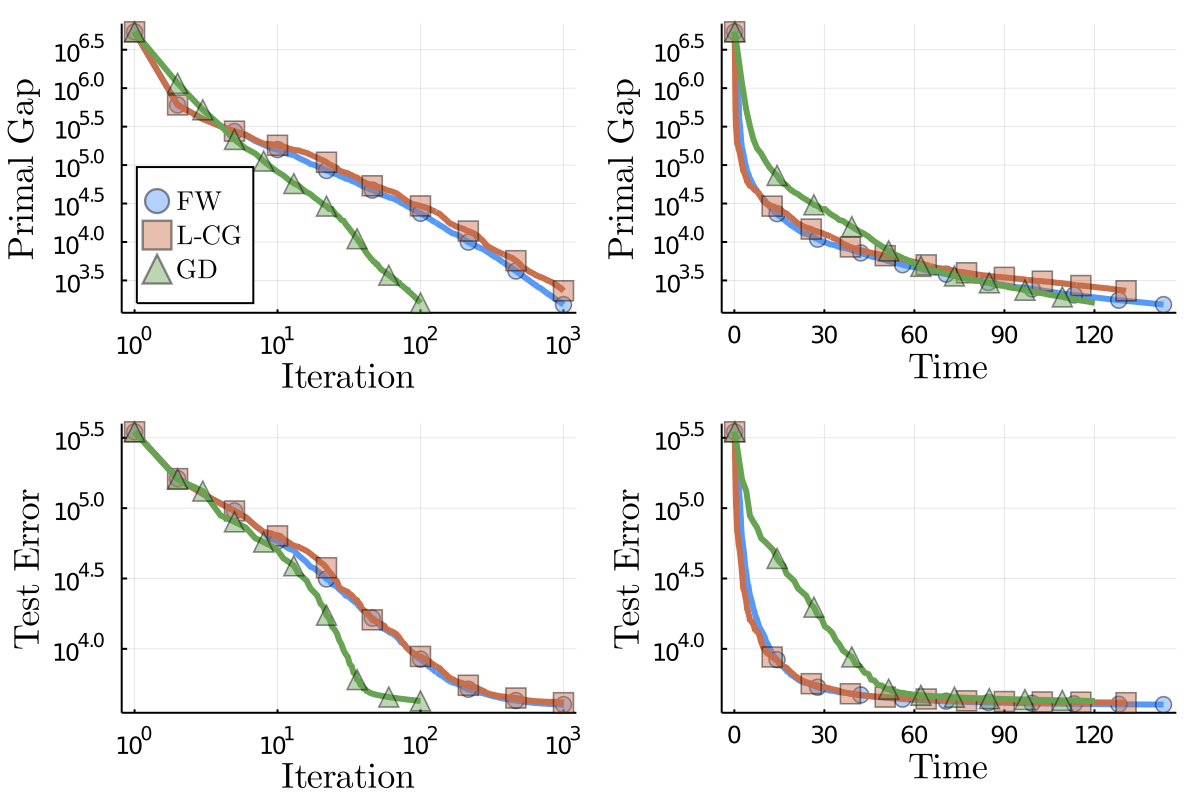Figure 2. Movielens results.

The results are presented in Figure 2. We can clearly observe that the computational cost of a single PGD iteration is much higher than the cost of a FW variant step. The FW variants tested complete $10^3$ iterations in around $120$ seconds, while the PGD algorithm only completes $10^2$ iterations in a similar time frame. We also observe that the progress per iteration made by each projection-free variant is smaller than the progress made by PGD, as expected. Note that, minimizing a linear function over the nuclear norm ball, in order to compute the LMO, amounts to computing the left and right singular vectors associated with the largest singular value, which we do using the $\texttt{ARPACK}$ Julia wrapper in the current example. On the other hand, projecting onto the nuclear norm ball requires computing a full singular value decomposition. The underlying linear solver can be switched by users developing their own LMO.

The top two figures in Figure 2 present the primal gap of the matrix completion problem objective function in terms of iteration count and wall-clock time. The two bottom figures show the performance on a test set of entries. Note that the test error stagnates for all methods, as expected. Even though the training error decreases linearly for PGD for all iterations, the test error stagnates quickly. The final test error of PGD is about $6\%$ higher than the final test error of the standard FW algorithm, which is also $2\%$ smaller than the final test error of the lazy FW algorithm. We would like to stress though that the intention here is primarily to showcase the algorithms and the results are considered to be illustrative in nature only rather than a proper evaluation with correct hyper-parameter tuning.

Another key aspect of FW algorithms is the sparsity of the provided solutions. Sparsity in this context refers to a matrix being low-rank. Although each solution is a dense matrix in terms of non-zeros, it can be decomposed as a sum of a small number of rank-one terms, each represented as a pair of left and right vectors. At each iteration, FW algorithms add at most one rank-one term to the iterate, thus resulting in a low-rank solution by design. In our example here, the final FW solution is of rank at most $95$ while the lazified version provides a sparser solution of rank at most $80$. The lower rank of the lazified FW is due to the fact that this algorithm sometimes avoids calling the LMO if there already exists an atom (here rank-1 factor) in the cache that guarantees enough progress; the higher sparsity might help with interpretability and robustness to noise. In contrast, the solution computed by PGD is of full column rank and even after truncating the spectrum, removing factors with small singular values, it is still of much higher rank than the FW solutions.

### Exact optimization with rational arithmetic

The package allows for exact optimization with rational arithmetic. For this, it suffices to set up the LMO to be rational and choose an appropriate step-size rule as detailed below. For the LMOs included in the package, this simply means initializing the radius with a rational-compatible element type, e.g., $\texttt{1}$, rather than a floating-point number, e.g., $\texttt{1.0}$. Given that numerators and denominators can become quite large in rational arithmetic, it is strongly advised to base the used rationals on extended-precision integer types such as $\texttt{BigInt}$, i.e., we use $\texttt{Rational{BigInt}}$. For the probability simplex LMO with a rational radius of $\texttt{1}$, the LMO would be created as follows:

lmo = FrankWolfe.ProbabilitySimplexOracle{Rational{BigInt}}(1)


As mentioned before, the second requirement ensuring that the computation runs in rational arithmetic is a rational-compatible step-size rule. The most basic step-size rule compatible with rational optimization is the $\texttt{agnostic}$ step-size rule with $\gamma_t = 2/(2+t)$. With this step-size rule, the gradient does not even need to be rational as long as the atom computed by the LMO is of a rational type. Assuming these requirements are met, all iterates and the computed solution will then be rational:

n = 100
x = fill(big(1)//100, n)
# equivalent to { 1/100 }^100


Another possible step-size rule is $\texttt{rationalshortstep}$ which computes the step size by minimizing the smoothness inequality as $\gamma_t = \frac{\langle \nabla f(\mathbf{x}_t), \mathbf{x}_t - \mathbf{v}_t\rangle}{2 L |\mathbf{x}_t - \mathbf{v}_t|^2}$. However, as this step size depends on an upper bound on the Lipschitz constant $L$ as well as the inner product with the gradient $\nabla f(\mathbf{x}_t)$, both have to be of a rational type.

### Doubly stochastic matrices

The set of doubly stochastic matrices or Birkhoff polytope appears in various combinatorial problems including matching and ranking. It is the convex hull of permutation matrices, a property of interest for FW algorithms because the individual atoms returned by the LMO only have $n$ non-zero entries for $n\times n$ matrices. A linear function can be minimized over the Birkhoff polytope using the Hungarian algorithm. This LMO is substantially more expensive than minimizing a linear function over the $\ell_1$-ball norm, and thus the algorithm performance benefits from lazification. We present the performance profile of several FW variants in the following example on $200\times 200$ matrices. The results are presented in Figure 3.

The per-iteration primal value evolution is nearly identical for FW and the lazy cache variants. We can observe a slower decrease rate in the first 10 iterations of BCG for both the primal value and the dual gap. This initial overhead is however compensated after the first iterations, BCG is the only algorithm terminating with the desired dual gap of $10^{-7}$ and not with the iteration limit. In terms of runtime, all lazified variants outperform the standard FW, the overhead of allocating and managing the cache are compensated by the reduced number of calls to the LMO.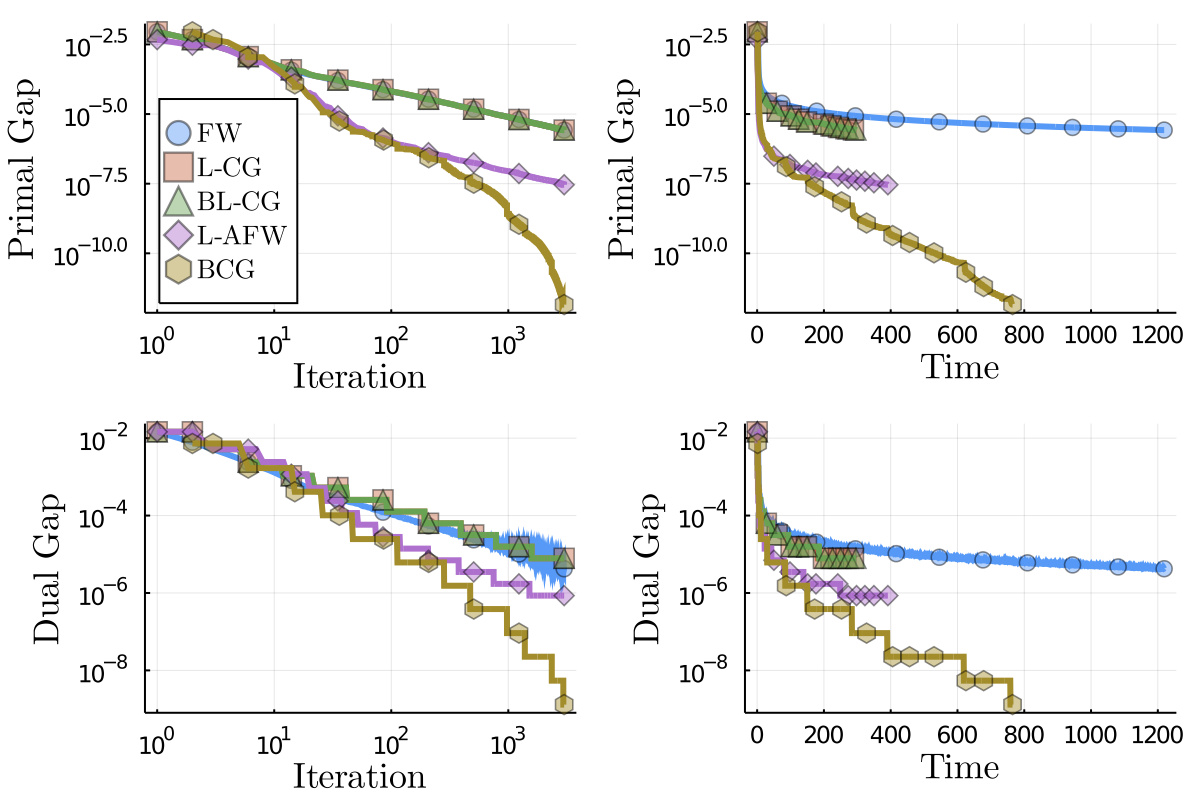Figure 3. Doubly stochastic matrices results.

### References

[FW] Frank, M., & Wolfe, P. (1956). An algorithm for quadratic programming. In Naval research logistics quarterly, 3(1-2), 95-110.

[LP] Levitin, E. S., & Polyak, B. T. (1966). Constrained minimization methods. In USSR Computational mathematics and mathematical physics, 6(5), 1-50.

[CP] Combettes, C. W., & Pokutta, S. (2021). Complexity of linear minimization and projection on some sets. In arXiv preprint arXiv:2101.10040. pdf

[BCP] Besançon, M., Carderera, A., & Pokutta, S. (2021). FrankWolfe.jl: a high-performance and flexible toolbox for Frank-Wolfe algorithms and Conditional Gradients. In arXiv preprint arXiv:2104.06675. pdf

[GM] Guélat, J., & Marcotte, P. (1986). Some comments on Wolfe’s ‘away step’. In Mathematical Programming 35(1) (pp. 110–119). Springer. pdf

[LJ] Lacoste-Julien, S., & Jaggi, M. (2015). On the global linear convergence of Frank-Wolfe optimization variants. In Advances in Neural Information Processing Systems 2015 (pp. 496-504). pdf

[BPZ] Braun, G., Pokutta, S., & Zink, D. (2017). Lazifying Conditional Gradient Algorithms. In Proceedings of the 34th International Conference on Machine Learning. pdf

[BPTW] Braun, G., Pokutta, S., Tu, D., & Wright, S. (2019). Blended conditonal gradients. In International Conference on Machine Learning (pp. 735-743). PMLR. pdf

[HL] Hazan, E. & Luo, H. (2016). Variance-reduced and projection-free stochastic optimization. In Proceedings of the 33rd International Conference on Machine Learning. pdf

[PNAM] Pedregosa, F., Negiar, G., Askari, A., & Jaggi, M. (2020). Linearly Convergent Frank-Wolfe with Backtracking Line-Search. In Proceedings of the 23rd International Conference on Artificial Intelligence and Statistics. pdf

]]>
Alejandro Carderera and Mathieu Besançon